# 1 本文写作背景

Hadley Wickham 因在统计应用领域做出的卓越贡献获得 2019 年考普斯会长奖（COPSS Presidents’ Award），这其中 ggplot2 可以说是厥功至伟。

2020 年左右，我得知tidytuesday项目，这个项目很有意思。

1. 找真实的数据，传播信息，开启话题。
2. 宣传 ggplot2 包和 dplyr 包，品牌营销。
3. 探索各种各样的可视化形式，给人启发。
4. 引人入坑 R 语言和数据可视化，壮大社区。

# 2 地震背景信息

• 1976 年河北唐山地震震级为 7.6 级
• 2008 年四川汶川地震震级为 8.0 级
• 2013 年四川雅安地震震级为 7.0 级
• 2021 年青海玛多地震震级为 7.4 级

# 3 1973-2010 年全球地震变化

## 3.1 数据准备

data(quake6, package = "MSG")
head(quake6)
##   Cat year month day   time    lat   long dep magnitude
## 1 PDE 1973     1   1 114238 -35.51 -16.21  33       6.0
## 2 PDE 1973     1   5 135429 -39.00 175.23 150       6.2
## 3 PDE 1973     1   6 155242 -14.66 166.38  36       6.1
## 4 PDE 1973     1  10 113227 -11.10 162.28  32       6.0
## 5 PDE 1973     1  15  90258  27.08 140.10 477       6.0
## 6 PDE 1973     1  18  92814  -6.87 149.99  43       6.8

str(quake6)
## 'data.frame':	4999 obs. of  9 variables:
##  $Cat : Factor w/ 3 levels "PDE","PDE-Q",..: 1 1 1 1 1 1 1 1 1 1 ... ##$ year     : int  1973 1973 1973 1973 1973 1973 1973 1973 1973 1973 ...
##  $month : int 1 1 1 1 1 1 1 1 1 1 ... ##$ day      : int  1 5 6 10 15 18 22 23 27 30 ...
##  $time : num 114238 135429 155242 113227 90258 ... ##$ lat      : num  -35.5 -39 -14.7 -11.1 27.1 ...
##  $long : num -16.2 175.2 166.4 162.3 140.1 ... ##$ dep      : int  33 150 36 32 477 43 33 97 55 43 ...
##  $magnitude: num 6 6.2 6.1 6 6 6.8 6.2 6.3 6 7.5 ... ## 3.2 震次趋势（年度） 下面先来看看每年地震次数变化趋势，如图3.1所示。 library(ggplot2) # 按年分组计数 aggregate(data = quake6, time ~ year, FUN = length) |> ggplot(aes(x = year, y = time)) + geom_point() + geom_line() + theme_classic() + theme(panel.grid.major.y = element_line(colour = "gray90")) + labs(x = "年份", y = "地震次数")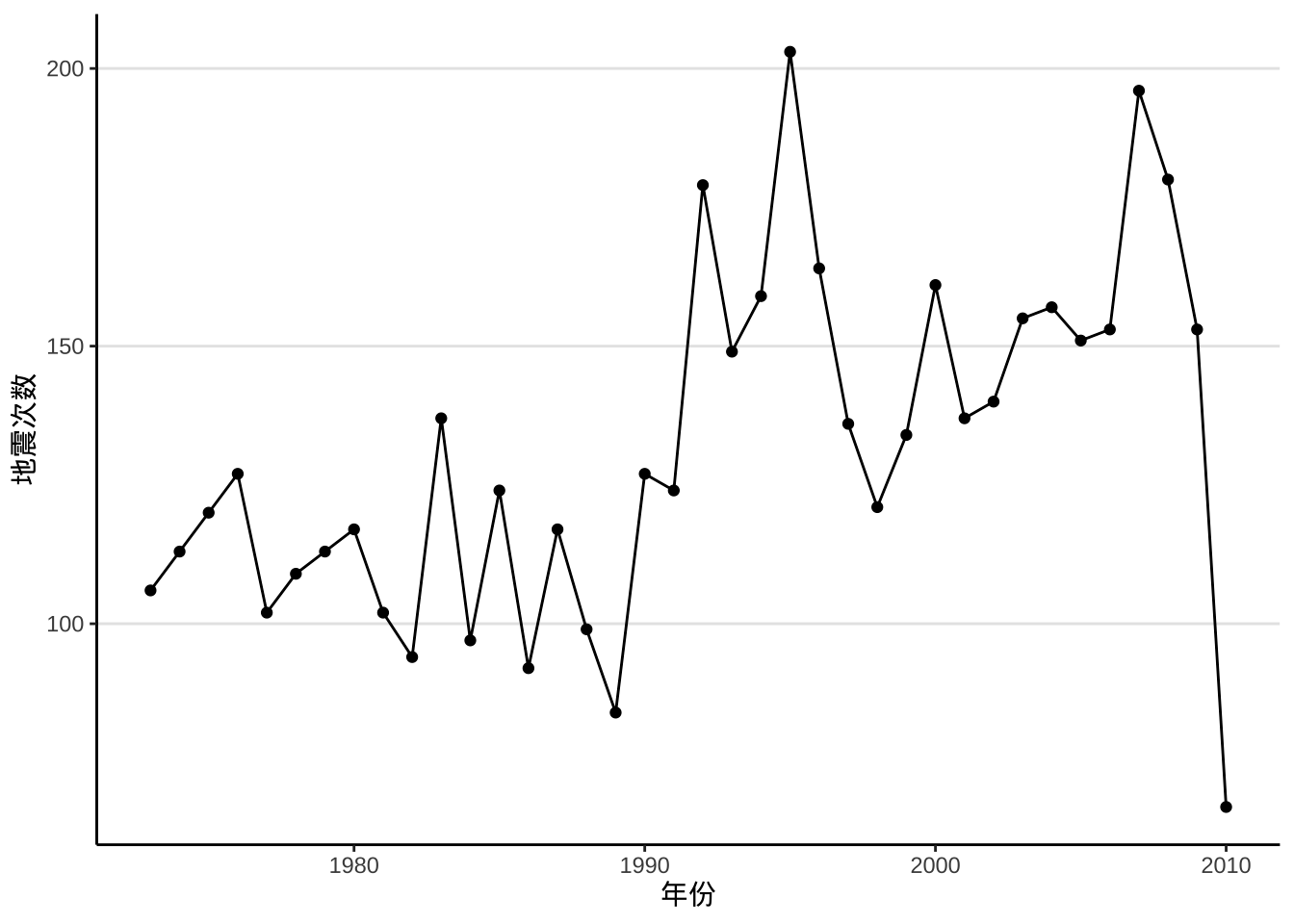图 3.1: 1973-2010 年世界 6级以上地震次数变化趋势 从图上来看，地震确实变多了。有坛友根据美国地震局发布的信息，解释此现象是因为探测能力变强了，能发现更多的地震，而不是地震变多了。 2010 年大家在论坛上讨论的时候，正是 4 月份，不是一年的累计量，因此图中看起来 2010 年的地震数量很少。 ## 3.3 震级分布（总体情况） 数据集 quake6 中记录的最大地震为 9 级，最小地震为 6 级。 range(quake6$magnitude)
##  6 9

ggplot(data = quake6, aes(x = magnitude)) +
geom_histogram(bins = 31, color = "gray40", fill = "gray90") +
geom_freqpoly(stat = "count") +
theme_minimal() +
labs(x = "震级", y = "次数")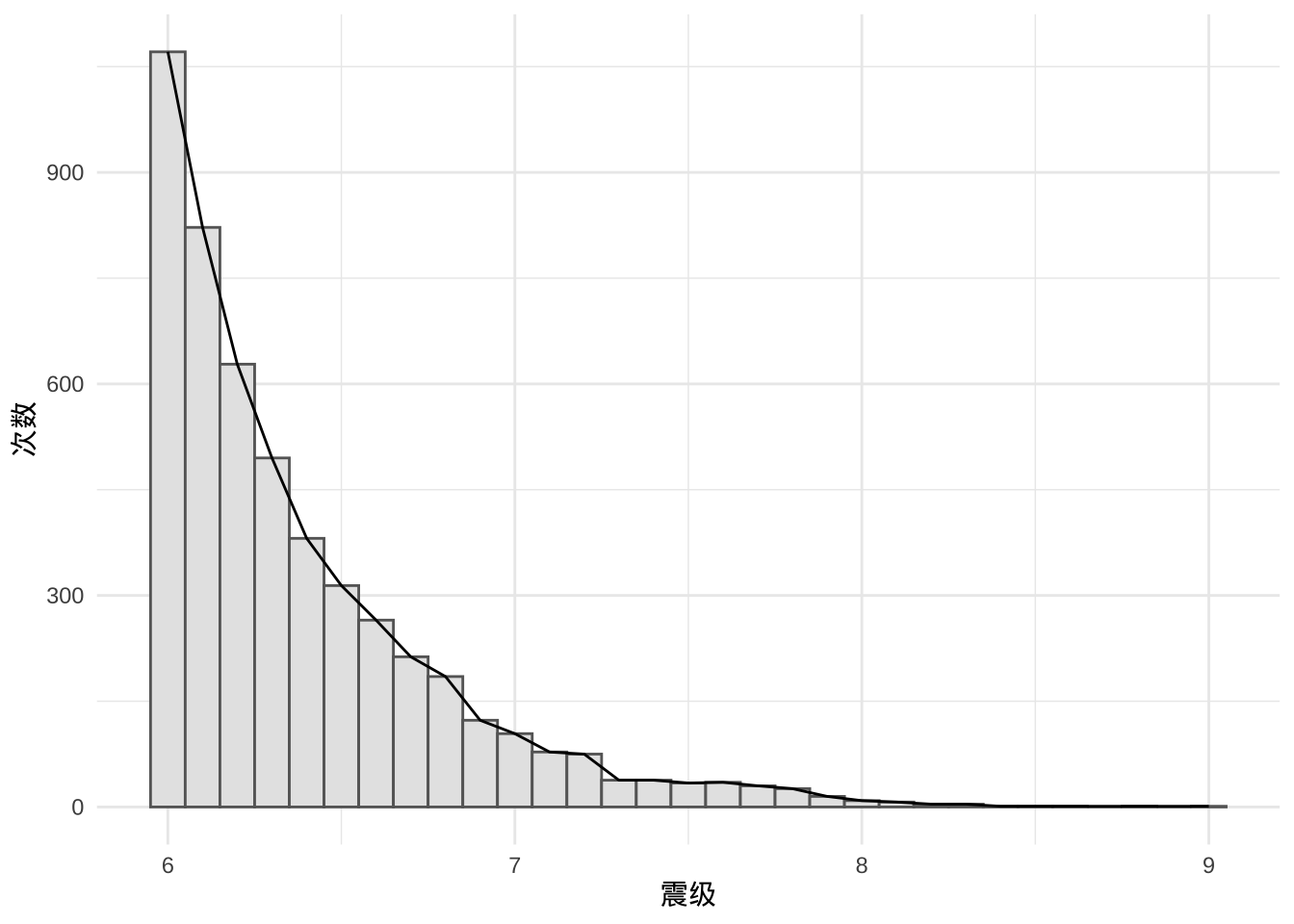## 3.4 震级分布（按年分组）

### 3.4.1 抖动图

library(ggbeeswarm)

ggplot(quake6, aes(x = as.factor(year), y = magnitude, colour = as.factor(year))) +
geom_quasirandom() +
coord_flip() +
geom_jitter() +
theme_minimal() +
theme(legend.position = "none") +
labs(y = "震级", x = "年份")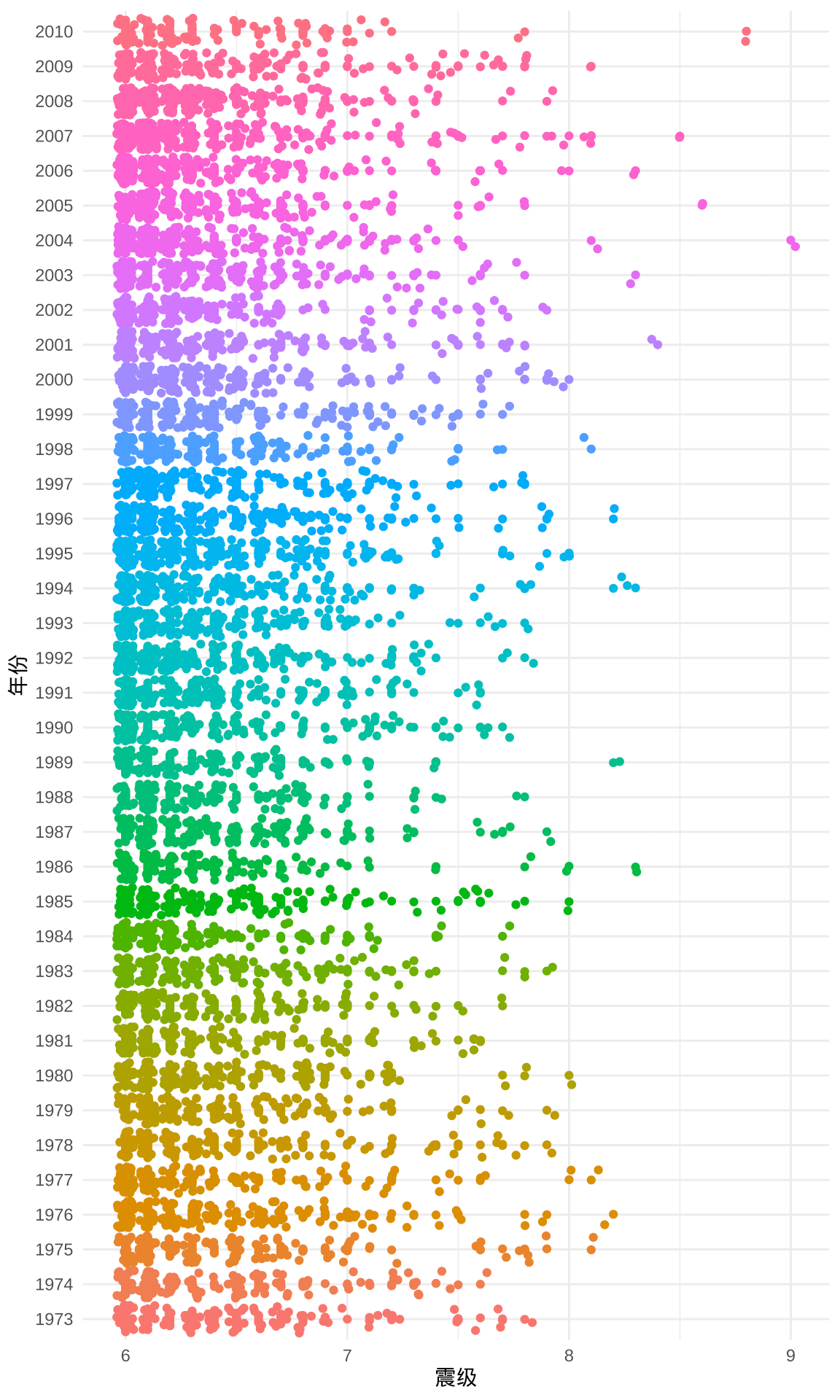### 3.4.2 岭线图

library(ggridges)
ggplot(quake6, aes(x = magnitude, y = as.factor(year), height = after_stat(density))) +
geom_ridgeline(stat = "density") +
theme_ridges() +
labs(x = "震级", y = "年份")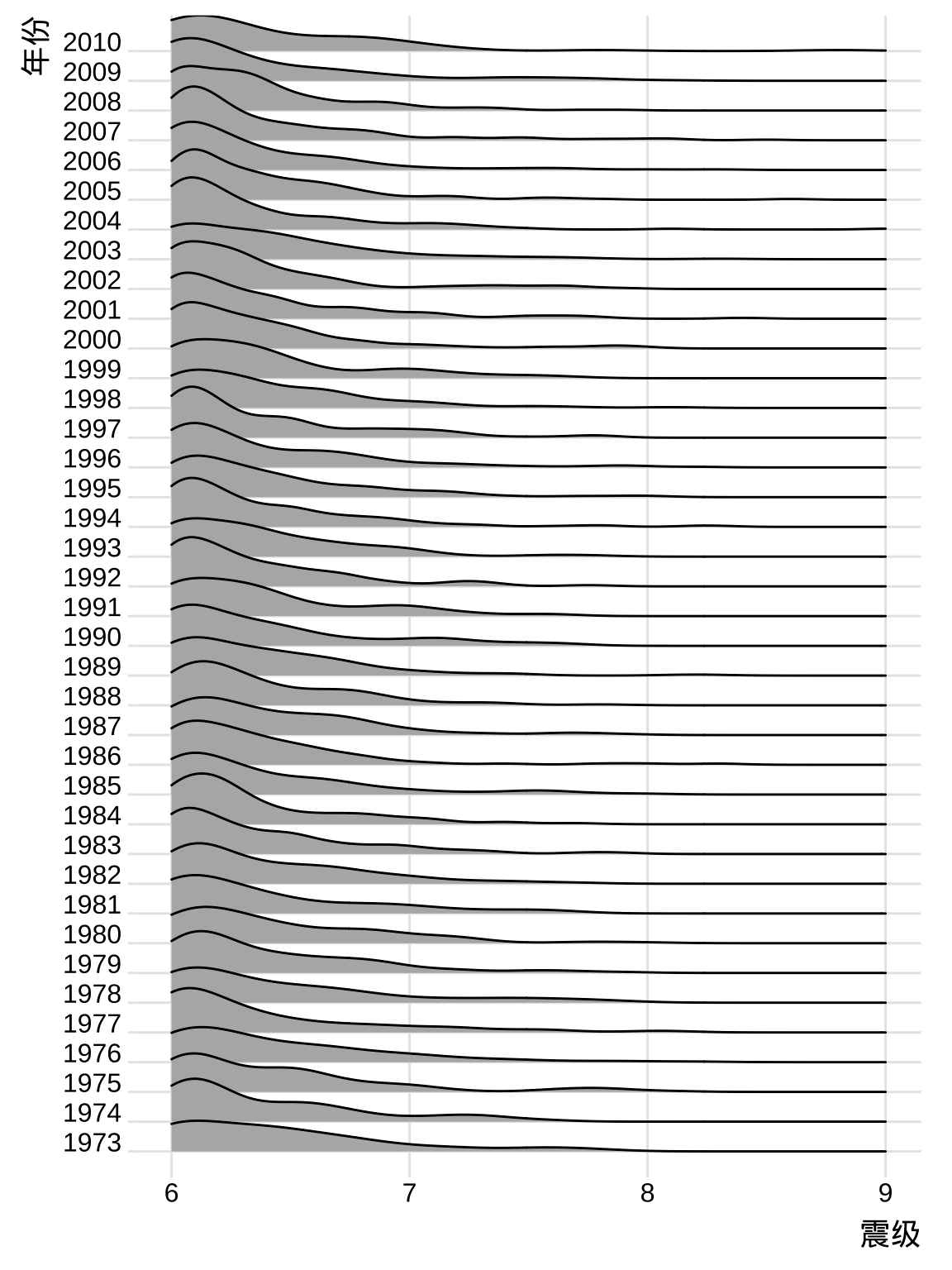### 3.4.3 葙线图

ggplot(quake6, aes(x = magnitude, y = as.factor(year))) +
geom_boxplot() +
theme_minimal() +
labs(x = "震级", y = "年份")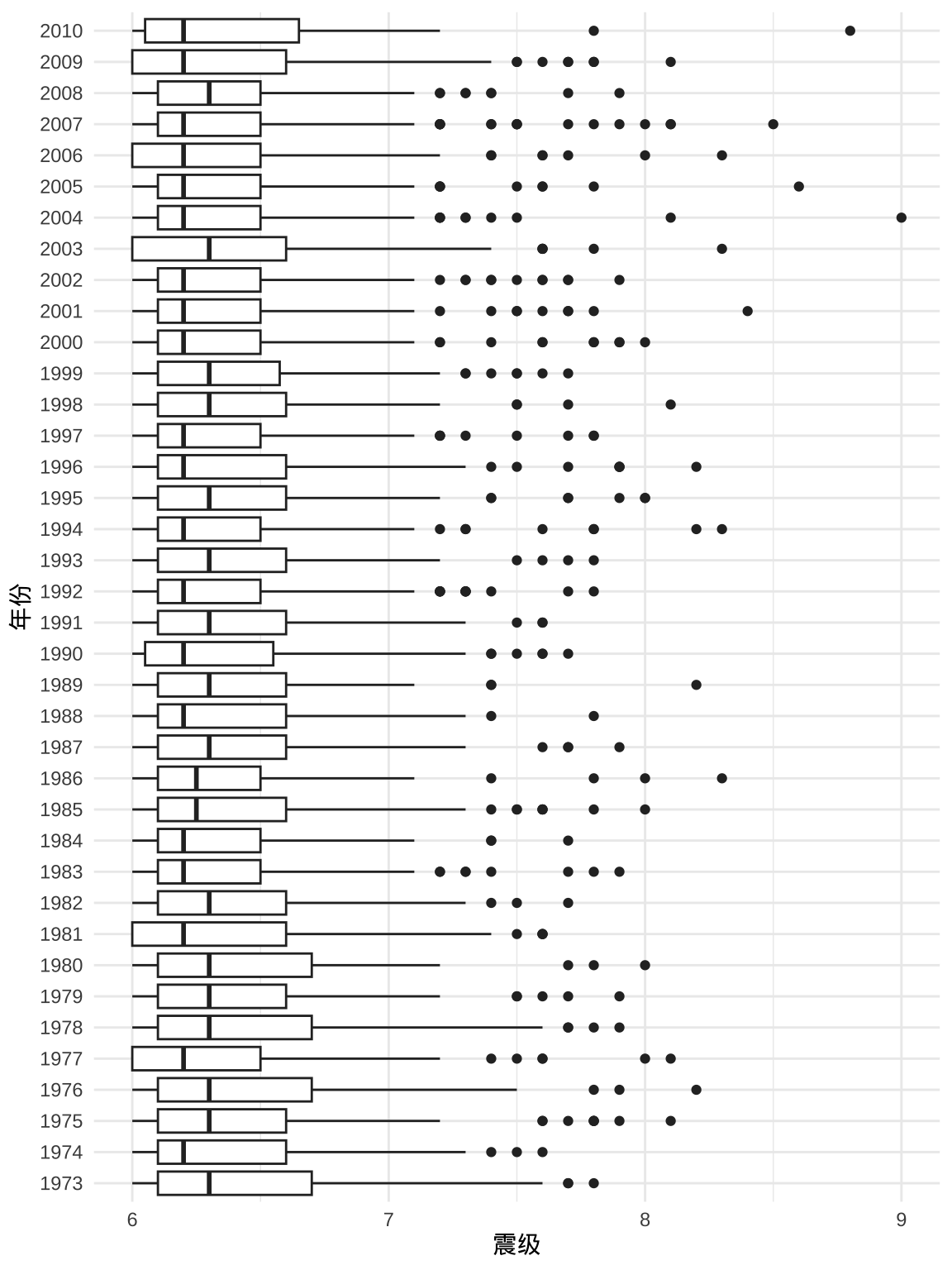### 3.4.4 提琴图

ggplot(quake6, aes(x = magnitude, y = as.factor(year))) +
geom_violin() +
theme_minimal() +
labs(x = "震级", y = "年份")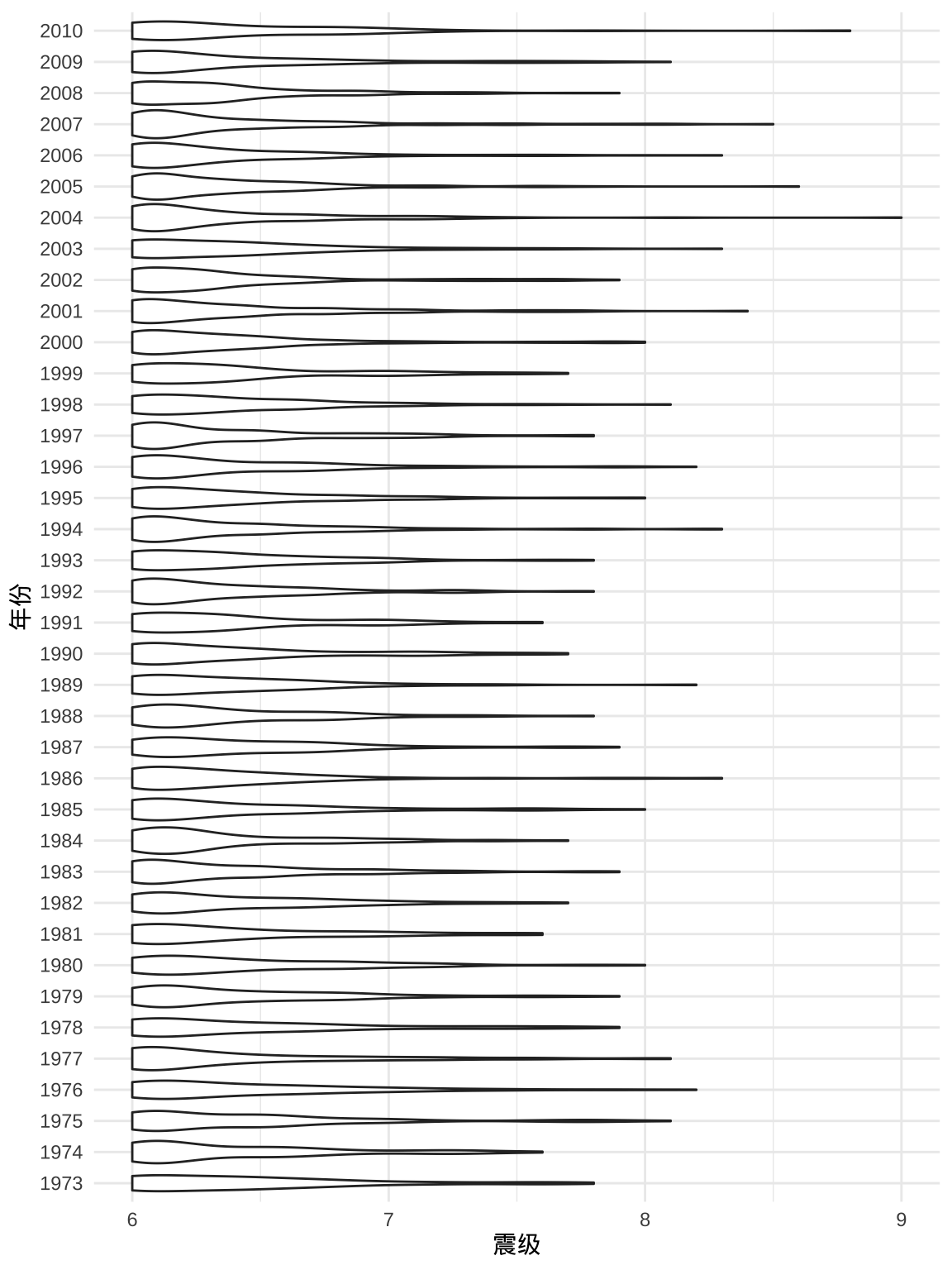## 3.5 震级分布（空间）

library(sf)
# 转为 sf 类型
quake6_sf <- st_as_sf(quake6, coords = c("long", "lat"), crs = 4326)
# 绘图
ggplot() +
geom_sf(data = quake6_sf, aes(color = magnitude), cex = .1) +
scale_color_viridis_c() +
theme_minimal() +
labs(
x = "经度", y = "纬度", color = "震级",
title = "1973-2010 年世界6级以上地震分布",
caption = "数据源：美国地震局"
)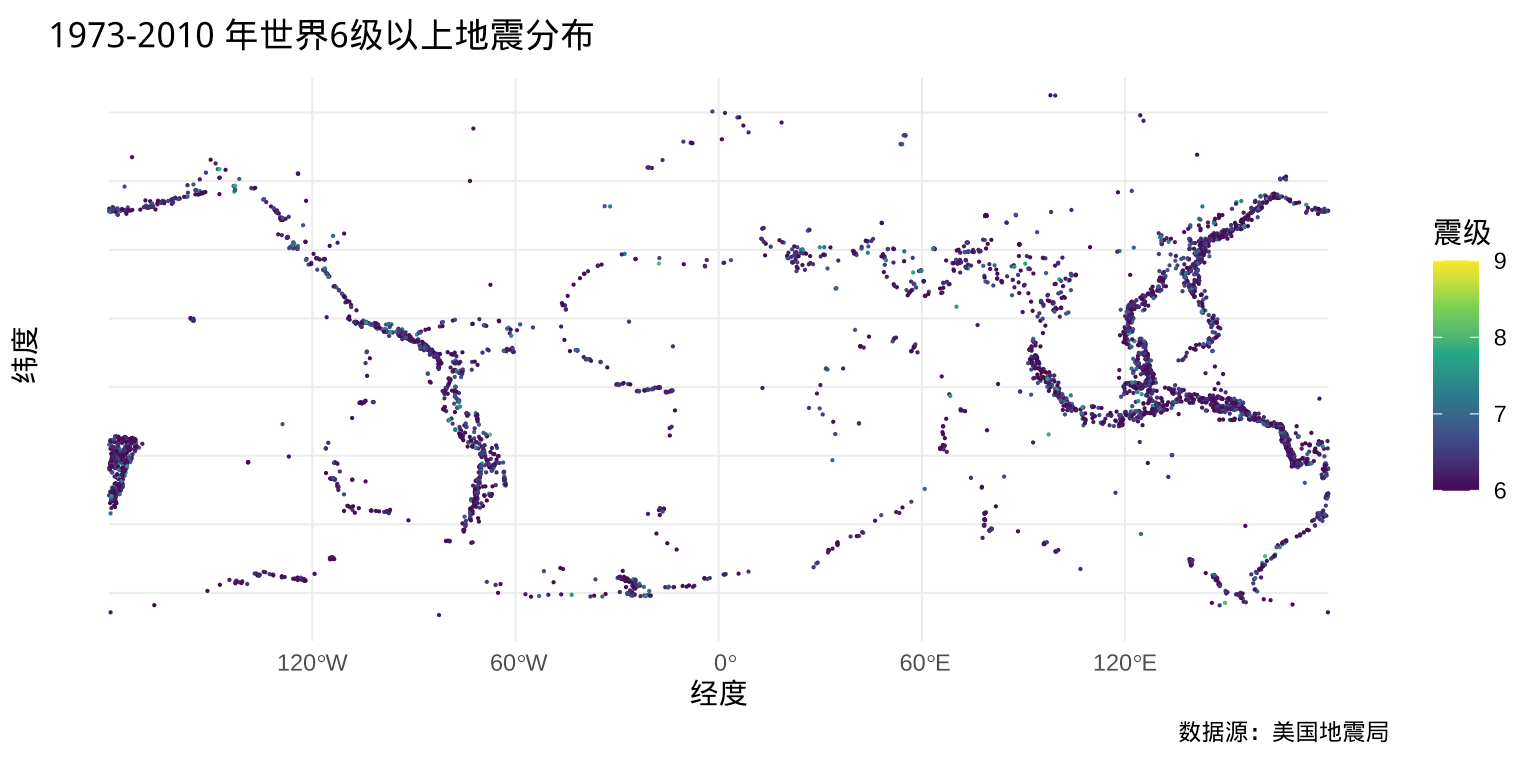# 4 2012-2022 年中国地震变化

## 4.1 数据准备

x <- read.delim(file = "data/quakes_20221231.xls", header = FALSE)

x[4:25, ]
##   "<Table>"
##   "<Column ss:Index=1 ss:AutoFitWidth=0 ss:Width=110/>"
##   "<Row>"
##   "<Cell><Data ss:Type=String>发震时刻</Data></Cell>"
##   "<Cell><Data ss:Type=String>震级(M)</Data></Cell>"
##   "<Cell><Data ss:Type=String>纬度(°)</Data></Cell>"
##   "<Cell><Data ss:Type=String>经度(°)</Data></Cell>"
##   "<Cell><Data ss:Type=String>深度(千米)</Data></Cell>"
##   "<Cell><Data ss:Type=String>参考位置</Data></Cell>"
##  "</Row>"
##  "<Row>"
##  "<Cell><Data ss:Type=String>2022-12-30 07:26:47</Data></Cell>"
##  "<Cell><Data ss:Type=Number>5.2</Data></Cell>"
##  "<Cell><Data ss:Type=String>-0.15</Data></Cell>"
##  "<Cell><Data ss:Type=Number>97.45</Data></Cell>"
##  "<Cell><Data ss:Type=Number>10</Data></Cell>"
##  "<Cell><Data ss:Type=String>印尼苏门答腊岛北部</Data></Cell>"
##  "</Row>"
##  "<Row>"
##  "<Cell><Data ss:Type=String>2022-12-29 21:13:36</Data></Cell>"
##  "<Cell><Data ss:Type=Number>5.2</Data></Cell>"
##  "<Cell><Data ss:Type=Number>6.10</Data></Cell>"

str_extract <- function(text, pattern, ...) regmatches(text, gregexpr(pattern, text, ...))

x_str <- str_extract(text = x$V1, pattern = "<Cell><Data ss:Type=(String|Number)>(.*?)</Data></Cell>") x_str <- unlist(x_str) head(x_str, 20) ##  "<Cell><Data ss:Type=String>发震时刻</Data></Cell>" ##  "<Cell><Data ss:Type=String>震级(M)</Data></Cell>" ##  "<Cell><Data ss:Type=String>纬度(°)</Data></Cell>" ##  "<Cell><Data ss:Type=String>经度(°)</Data></Cell>" ##  "<Cell><Data ss:Type=String>深度(千米)</Data></Cell>" ##  "<Cell><Data ss:Type=String>参考位置</Data></Cell>" ##  "<Cell><Data ss:Type=String>2022-12-30 07:26:47</Data></Cell>" ##  "<Cell><Data ss:Type=Number>5.2</Data></Cell>" ##  "<Cell><Data ss:Type=String>-0.15</Data></Cell>" ##  "<Cell><Data ss:Type=Number>97.45</Data></Cell>" ##  "<Cell><Data ss:Type=Number>10</Data></Cell>" ##  "<Cell><Data ss:Type=String>印尼苏门答腊岛北部</Data></Cell>" ##  "<Cell><Data ss:Type=String>2022-12-29 21:13:36</Data></Cell>" ##  "<Cell><Data ss:Type=Number>5.2</Data></Cell>" ##  "<Cell><Data ss:Type=Number>6.10</Data></Cell>" ##  "<Cell><Data ss:Type=Number>126.25</Data></Cell>" ##  "<Cell><Data ss:Type=Number>120</Data></Cell>" ##  "<Cell><Data ss:Type=String>菲律宾棉兰老岛</Data></Cell>" ##  "<Cell><Data ss:Type=String>2022-12-29 01:16:36</Data></Cell>" ##  "<Cell><Data ss:Type=Number>5.4</Data></Cell>" 去掉多余的字符，比如成对的 <Cell></Cell>，留下想要的数据字段。 x_str_tidy <- gsub(x = x_str, pattern = "<Cell><Data ss:Type=(String|Number)>(.*?)</Data></Cell>", replacement = "\\2") head(x_str_tidy, 20) ##  "发震时刻" "震级(M)" "纬度(°)" ##  "经度(°)" "深度(千米)" "参考位置" ##  "2022-12-30 07:26:47" "5.2" "-0.15" ##  "97.45" "10" "印尼苏门答腊岛北部" ##  "2022-12-29 21:13:36" "5.2" "6.10" ##  "126.25" "120" "菲律宾棉兰老岛" ##  "2022-12-29 01:16:36" "5.4" 可以说，这种办法简直是把 xls 文件给砸碎了，万幸地是数据文件还可以被当作 XML 文件读取，因此只要 xls 文件坏得还不彻底，这办法总是可以用的。 那么接下来就要拼起来，拼成一个 matrix，每一行记录包含 6 个字段。字符串向量 x_str_tidy 开头 6 个元素是表头，余下的 x_str_tidy[-c(1:6)] 是观测值，长度为 65304，分成 6 列，一共 10884 行。 dat <- matrix( data = x_str_tidy[-c(1:6)], ncol = 6, byrow = TRUE, dimnames = list(c(1:(length(x_str_tidy) / 6 - 1)), x_str_tidy[1:6]) ) head(dat) ## 发震时刻 震级(M) 纬度(°) 经度(°) 深度(千米) ## 1 "2022-12-30 07:26:47" "5.2" "-0.15" "97.45" "10" ## 2 "2022-12-29 21:13:36" "5.2" "6.10" "126.25" "120" ## 3 "2022-12-29 01:16:36" "5.4" "41.88" "79.56" "10" ## 4 "2022-12-29 00:34:20" "6.0" "-21.30" "171.45" "10" ## 5 "2022-12-28 21:19:22" "3.6" "40.16" "83.12" "100" ## 6 "2022-12-28 16:45:12" "3.0" "38.88" "75.89" "35" ## 参考位置 ## 1 "印尼苏门答腊岛北部" ## 2 "菲律宾棉兰老岛" ## 3 "吉尔吉斯斯坦" ## 4 "洛亚蒂群岛东南" ## 5 "新疆阿克苏地区沙雅县" ## 6 "新疆克孜勒苏州阿克陶县" 毕竟，不同列的数据类型不一样，需要单独处理，而 matrix 要求各列类型一致，为方便后续数据操作，将数据集转为 data.frame 类型。 dat <- as.data.frame(dat, check.names = FALSE, stringsAsFactors = FALSE) 查看初步整理出来的数据。 head(dat) ## 发震时刻 震级(M) 纬度(°) 经度(°) 深度(千米) 参考位置 ## 1 2022-12-30 07:26:47 5.2 -0.15 97.45 10 印尼苏门答腊岛北部 ## 2 2022-12-29 21:13:36 5.2 6.10 126.25 120 菲律宾棉兰老岛 ## 3 2022-12-29 01:16:36 5.4 41.88 79.56 10 吉尔吉斯斯坦 ## 4 2022-12-29 00:34:20 6.0 -21.30 171.45 10 洛亚蒂群岛东南 ## 5 2022-12-28 21:19:22 3.6 40.16 83.12 100 新疆阿克苏地区沙雅县 ## 6 2022-12-28 16:45:12 3.0 38.88 75.89 35 新疆克孜勒苏州阿克陶县 ## 4.2 数据操作 根据字段的实际类型，首先做一些基本的类型转换，如下： dat <- within(dat, { 发震时刻 <- as.POSIXlt(发震时刻, format = "%Y-%m-%d %H:%M:%S", tz = "UTC") 震级(M) <- as.numeric(震级(M)) 纬度(°) <- as.numeric(纬度(°)) 经度(°) <- as.numeric(经度(°)) 深度(千米) <- as.numeric(深度(千米)) }) 从「发震时刻」字段提取日期、年份、月份、发震时段等信息。 dat <- within(dat, { 日期 <- format(发震时刻, format = "%Y-%m-%d", tz = "UTC") 年份 <- format(发震时刻, format = "%Y", tz = "UTC") 月份 <- format(发震时刻, format = "%m", tz = "UTC") 时段 <- format(发震时刻, format = "%H", tz = "UTC") }) 整理好的数据集 dat 如下： str(dat) ## 'data.frame': 10884 obs. of 10 variables: ##$ 发震时刻  : POSIXlt, format: "2022-12-30 07:26:47" "2022-12-29 21:13:36" ...
##  $震级(M) : num 5.2 5.2 5.4 6 3.6 3 3 4.1 4 5.2 ... ##$ 纬度(°)   : num  -0.15 6.1 41.88 -21.3 40.16 ...
##  $经度(°) : num 97.5 126.2 79.6 171.4 83.1 ... ##$ 深度(千米): num  10 120 10 10 100 35 111 10 10 10 ...
##  $参考位置 : chr "印尼苏门答腊岛北部" "菲律宾棉兰老岛" "吉尔吉斯斯坦" "洛亚蒂群岛东南" ... ##$ 时段      : chr  "07" "21" "01" "00" ...
##  $月份 : chr "12" "12" "12" "12" ... ##$ 年份      : chr  "2022" "2022" "2022" "2022" ...
##  \$ 日期      : chr  "2022-12-30" "2022-12-29" "2022-12-29" "2022-12-29" ...

## 4.3 数据探索

### 4.3.1 震次分布（棋盘图）

aggregate(data = dat, 参考位置 ~ 年份 + 月份, FUN = length) |>
ggplot(aes(x = 年份, y = 月份, size = 参考位置)) +
geom_point() +
theme_minimal() +
labs(size = "次数")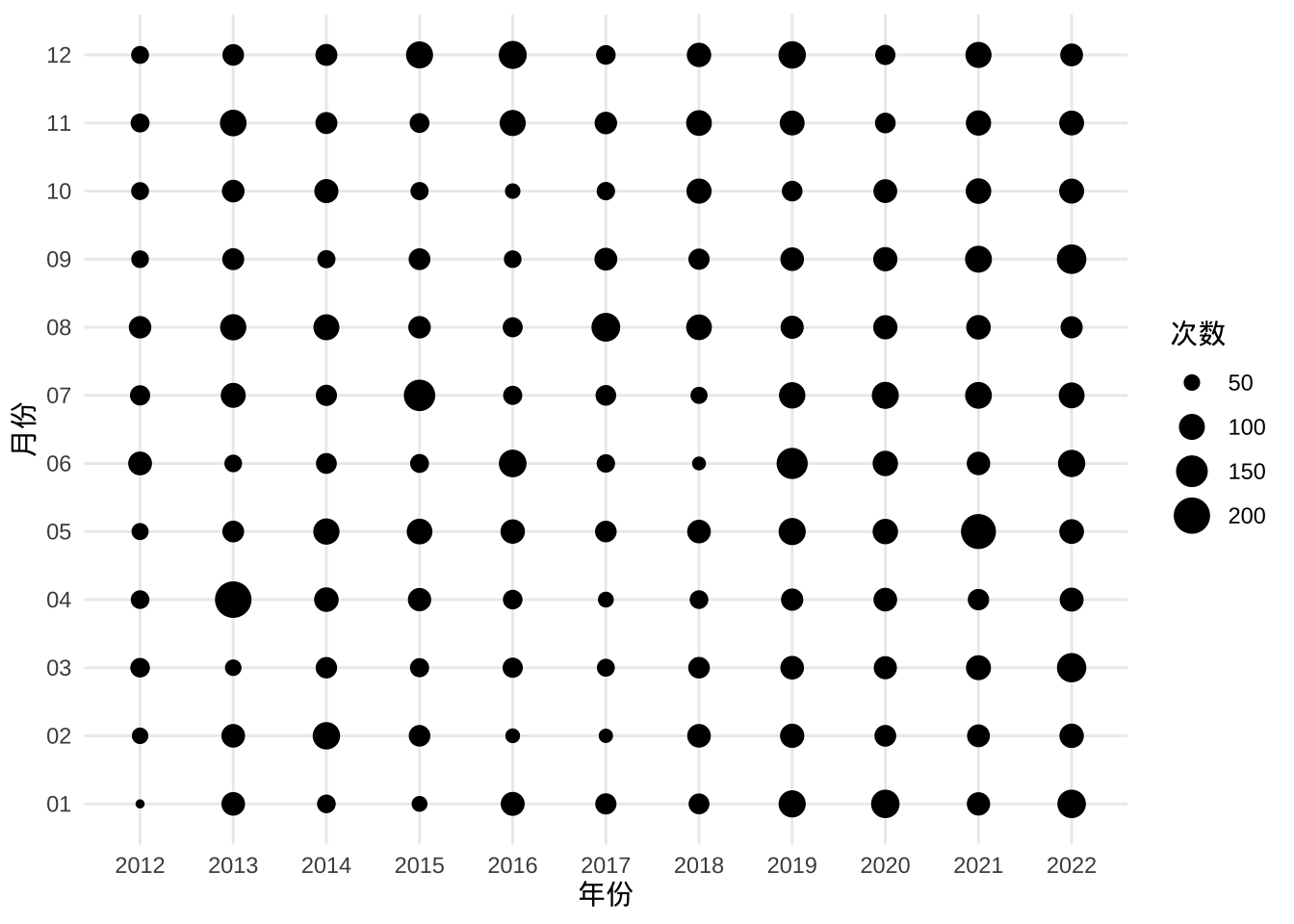aggregate(
data = subset(dat, subset = 震级(M) > 4.5),
参考位置 ~ 年份 + 月份, FUN = length
) |>
ggplot(aes(x = 年份, y = 月份, size = 参考位置)) +
geom_point() +
theme_minimal() +
labs(size = "次数")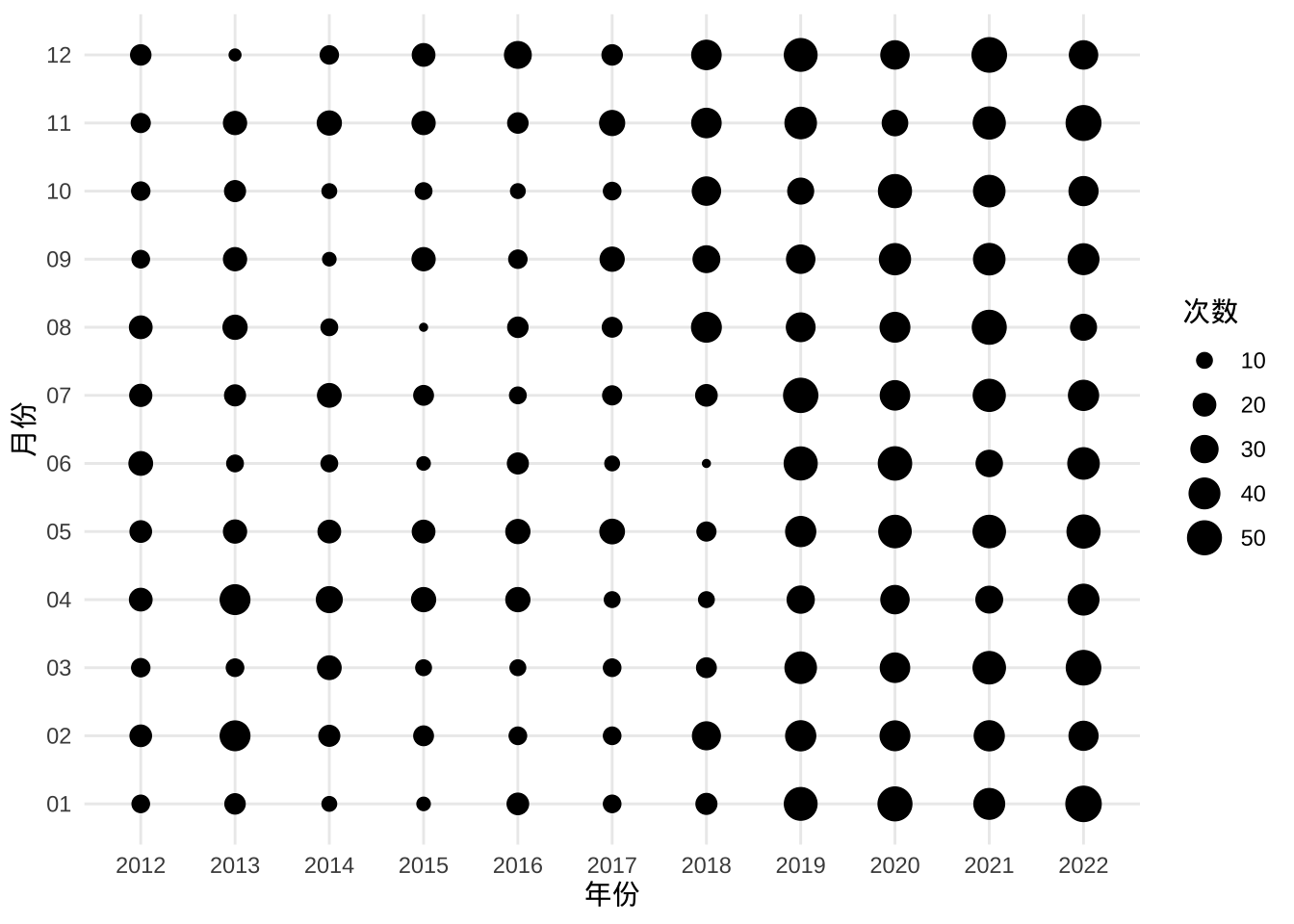aggregate(
data = subset(dat, subset = 震级(M) >= 6),
参考位置 ~ 年份 + 月份, FUN = length
) |>
ggplot(aes(x = 年份, y = 月份, size = 参考位置)) +
geom_point() +
theme_minimal() +
labs(size = "次数")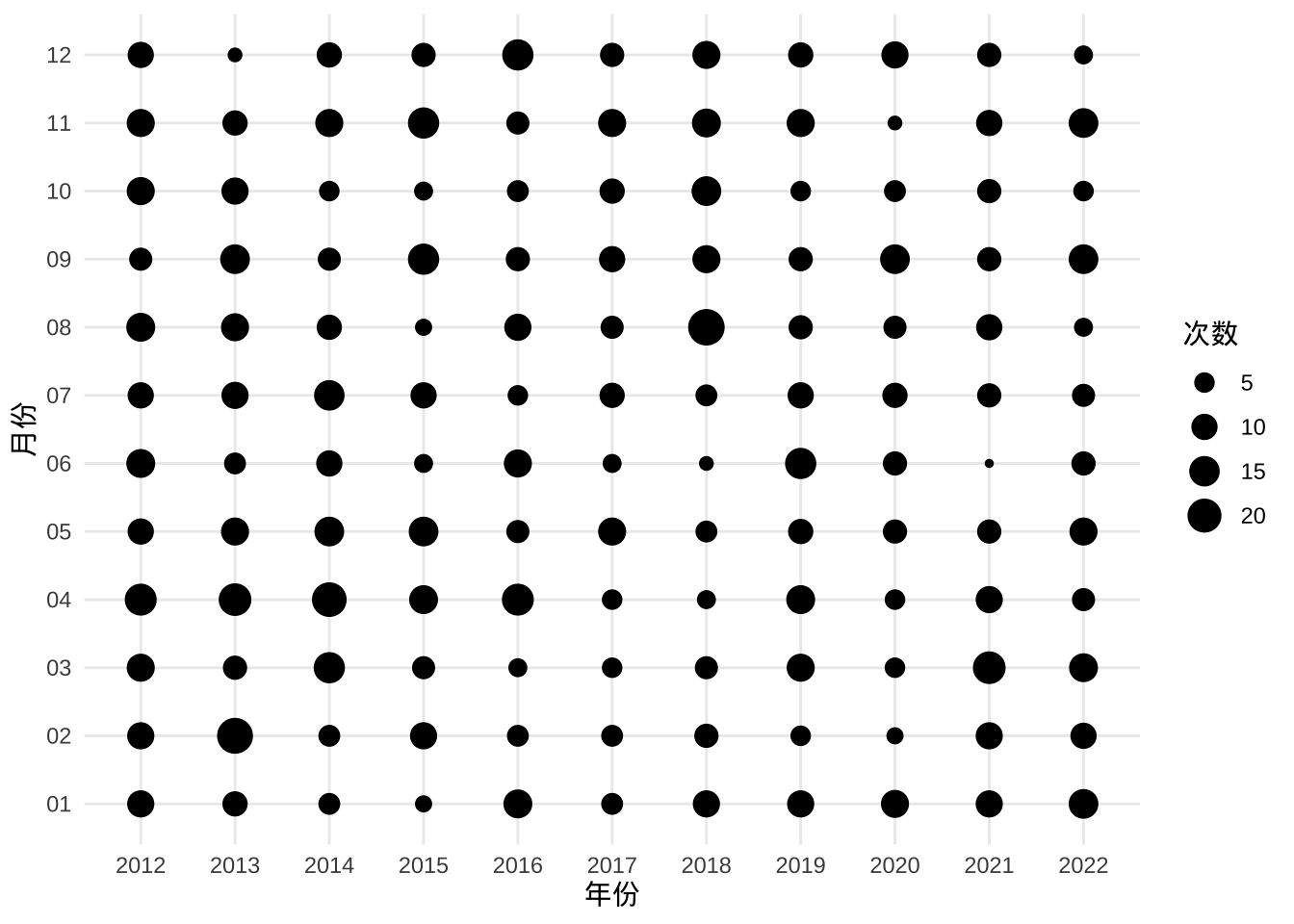### 4.3.2 震级分布（直方图）

ggplot(data = dat, aes(x = 震级(M), y = after_stat(count), fill = 年份)) +
geom_histogram(binwidth = 0.2) +
theme_minimal() +
labs(y = "地震次数")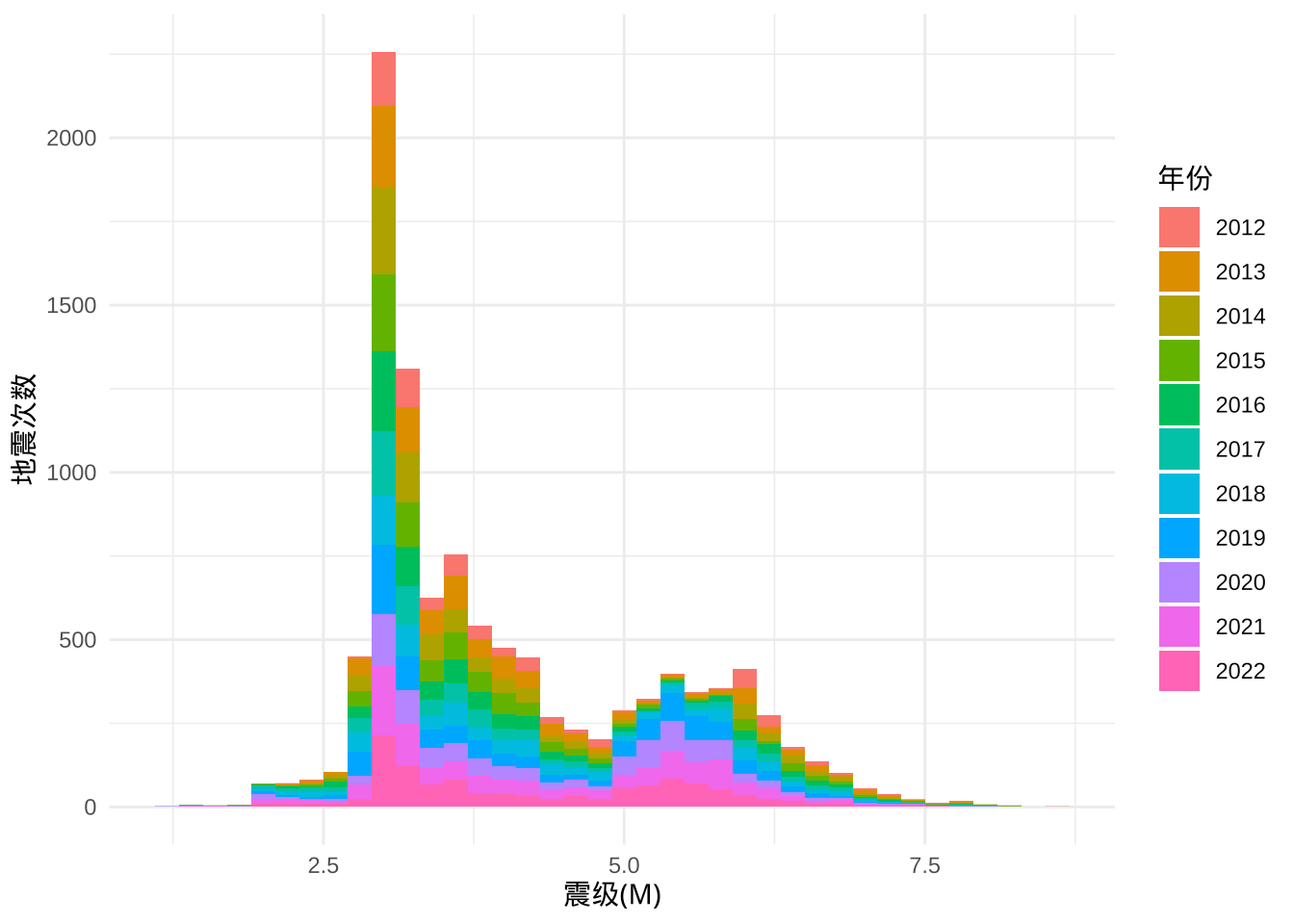### 4.3.3 震级分布（抖动图）

ggplot(dat, aes(x = 年份, y = 震级(M), color = 年份)) +
geom_quasirandom() +
coord_flip() +
geom_jitter() +
theme_minimal()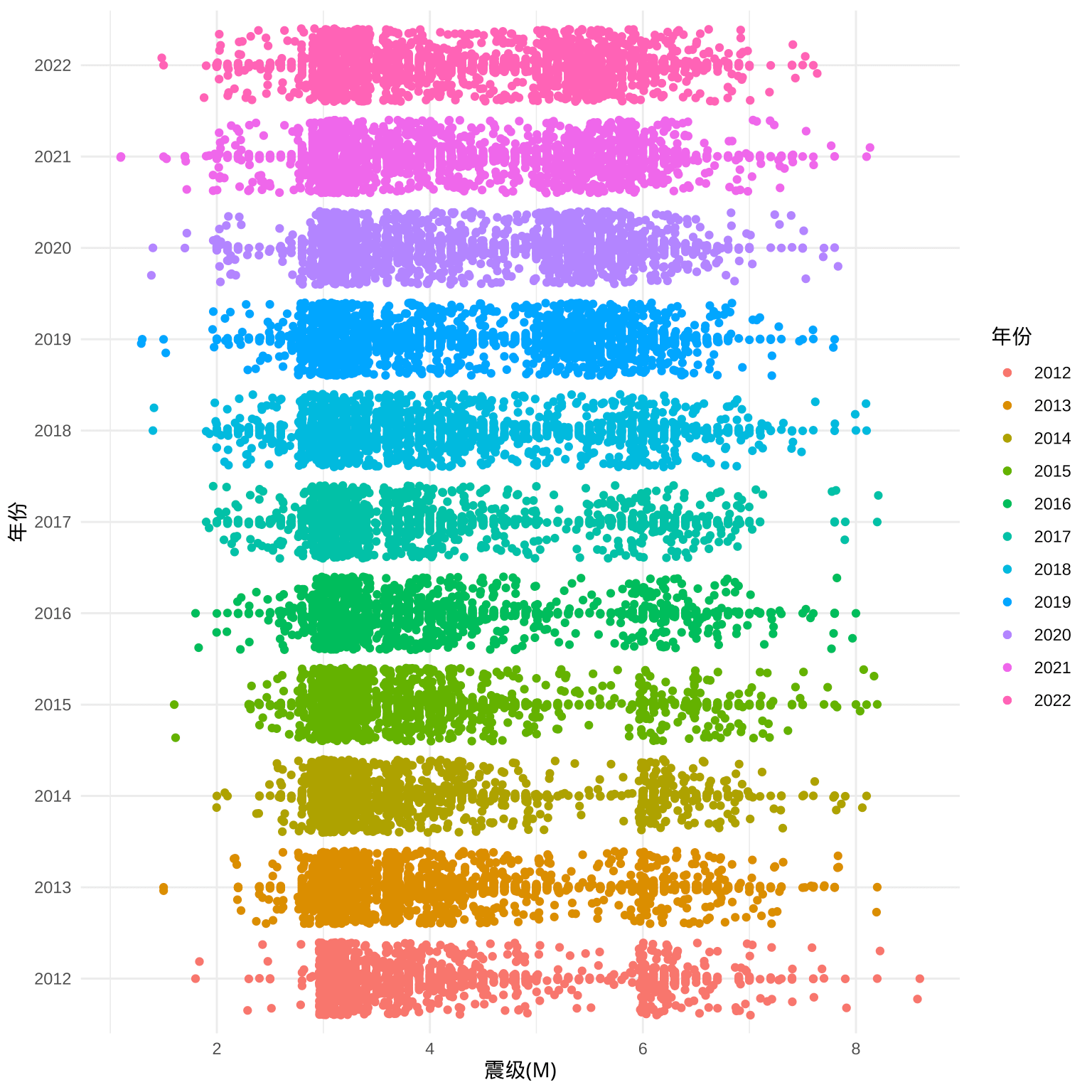### 4.3.4 震级分布（岭线图）

ggplot(dat, aes(x = 震级(M), y = 年份, fill = 年份)) +
geom_density_ridges(show.legend = FALSE) +
theme_ridges()
## Picking joint bandwidth of 0.246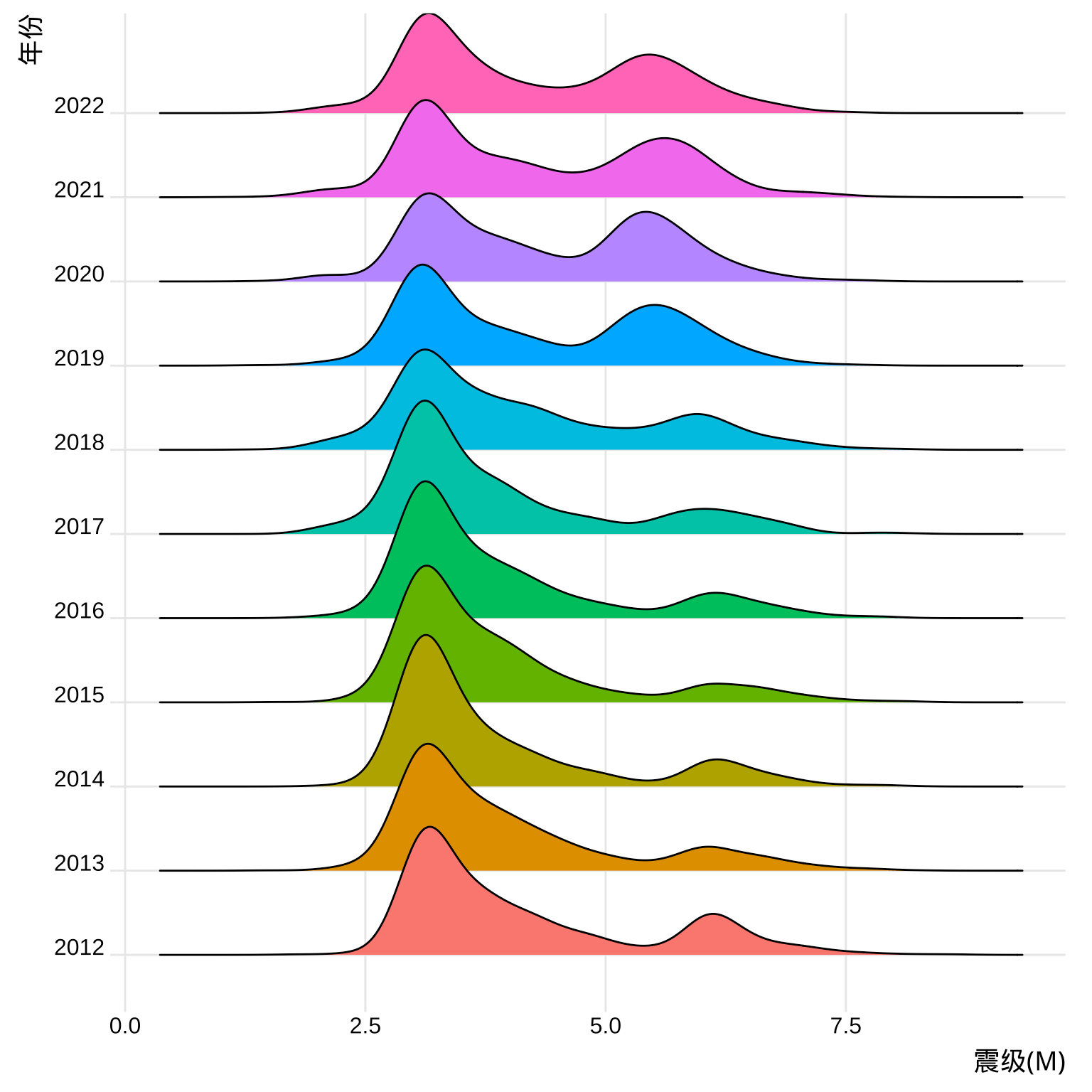subset(dat, subset = 震级(M) > 4.5) |>
ggplot(aes(x = 震级(M), y = 年份, fill = 年份)) +
geom_density_ridges(show.legend = FALSE) +
theme_ridges()
## Picking joint bandwidth of 0.194## 4.4 数据分析

### 4.4.1 震级的空间分布

library(sf)
# data.frame 转为 sf
dat_sf <- st_as_sf(dat, coords = c("经度(°)", "纬度(°)"), crs = 4326)
# 查看数据
dat_sf
## Simple feature collection with 10884 features and 8 fields
## Geometry type: POINT
## Dimension:     XY
## Bounding box:  xmin: -180 ymin: -69.9 xmax: 179.8 ymax: 86.94
## Geodetic CRS:  WGS 84
## First 10 features:
##               发震时刻 震级(M) 深度(千米)               参考位置 时段 月份 年份
## 1  2022-12-30 07:26:47     5.2         10     印尼苏门答腊岛北部   07   12 2022
## 2  2022-12-29 21:13:36     5.2        120         菲律宾棉兰老岛   21   12 2022
## 3  2022-12-29 01:16:36     5.4         10           吉尔吉斯斯坦   01   12 2022
## 4  2022-12-29 00:34:20     6.0         10         洛亚蒂群岛东南   00   12 2022
## 5  2022-12-28 21:19:22     3.6        100   新疆阿克苏地区沙雅县   21   12 2022
## 6  2022-12-28 16:45:12     3.0         35 新疆克孜勒苏州阿克陶县   16   12 2022
## 7  2022-12-27 20:28:22     3.0        111     新疆喀什地区叶城县   20   12 2022
## 8  2022-12-27 11:30:10     4.1         10       青海海北州门源县   11   12 2022
## 9  2022-12-27 06:56:20     4.0         10         台湾花莲县海域   06   12 2022
## 10 2022-12-26 20:21:09     5.2         10             埃塞俄比亚   20   12 2022
##          日期            geometry
## 1  2022-12-30 POINT (97.45 -0.15)
## 2  2022-12-29   POINT (126.2 6.1)
## 3  2022-12-29 POINT (79.56 41.88)
## 4  2022-12-29 POINT (171.4 -21.3)
## 5  2022-12-28 POINT (83.12 40.16)
## 6  2022-12-28 POINT (75.89 38.88)
## 7  2022-12-27 POINT (76.27 37.32)
## 8  2022-12-27 POINT (101.3 37.74)
## 9  2022-12-27 POINT (122.5 23.84)
## 10 2022-12-26   POINT (40.1 14.5)

ggplot() +
geom_sf(data = dat_sf, aes(color = 震级(M)), cex = .1) +
scale_color_viridis_c() +
theme_minimal() +
labs(
title = "2012-01-01 至 2022-12-31 的地震分布",
caption = "数据源：中国地震台网"
)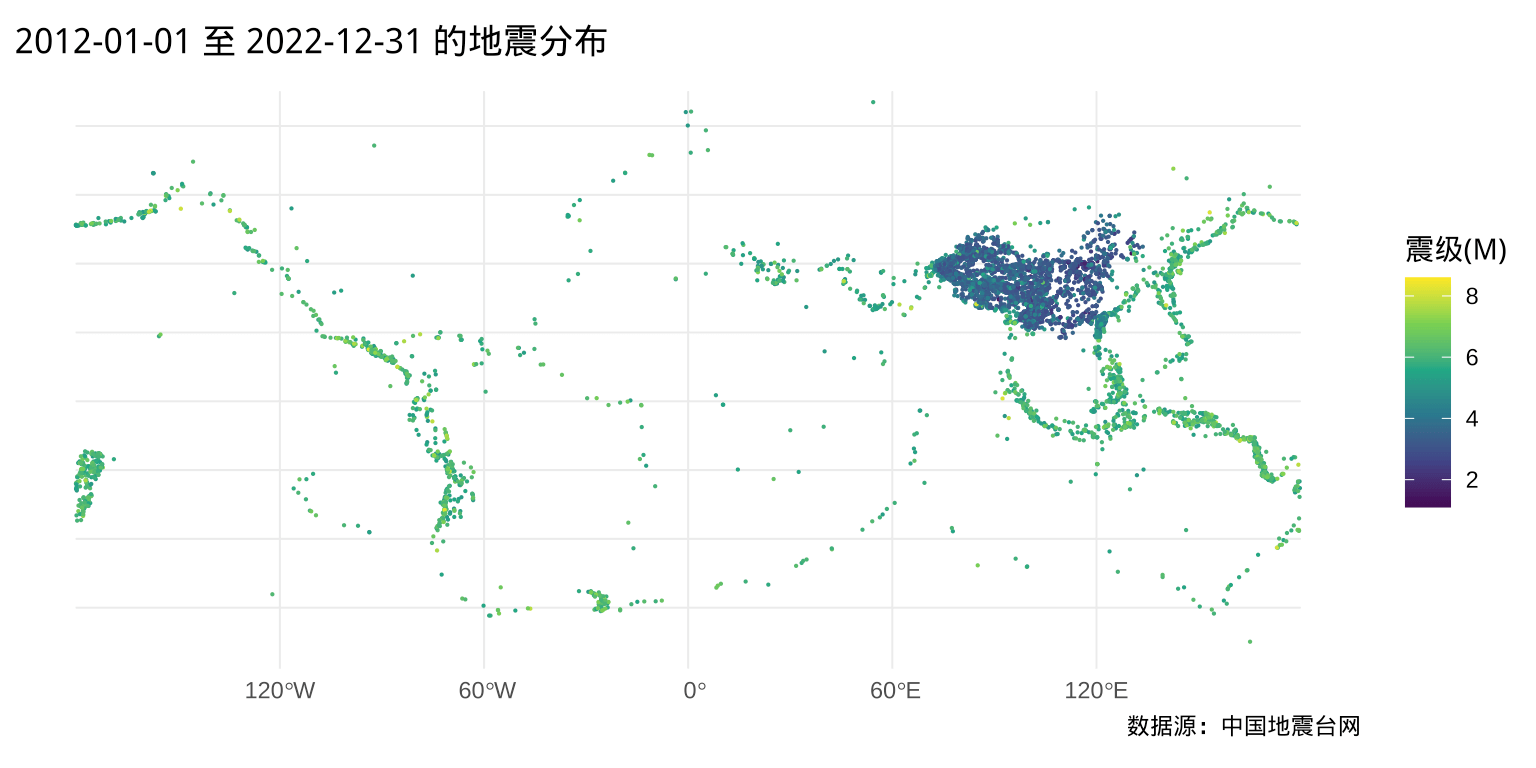ggplot() +
geom_sf(data = dat_sf, aes(color = 震级(M)), cex = .1) +
scale_color_viridis_c() +
coord_sf(xlim = c(73.68, 135.2), ylim = c(3.984, 53.65)) +
theme_minimal() +
labs(
title = "2012-01-01 至 2022-12-31 的地震分布",
caption = "数据源：中国地震台网"
)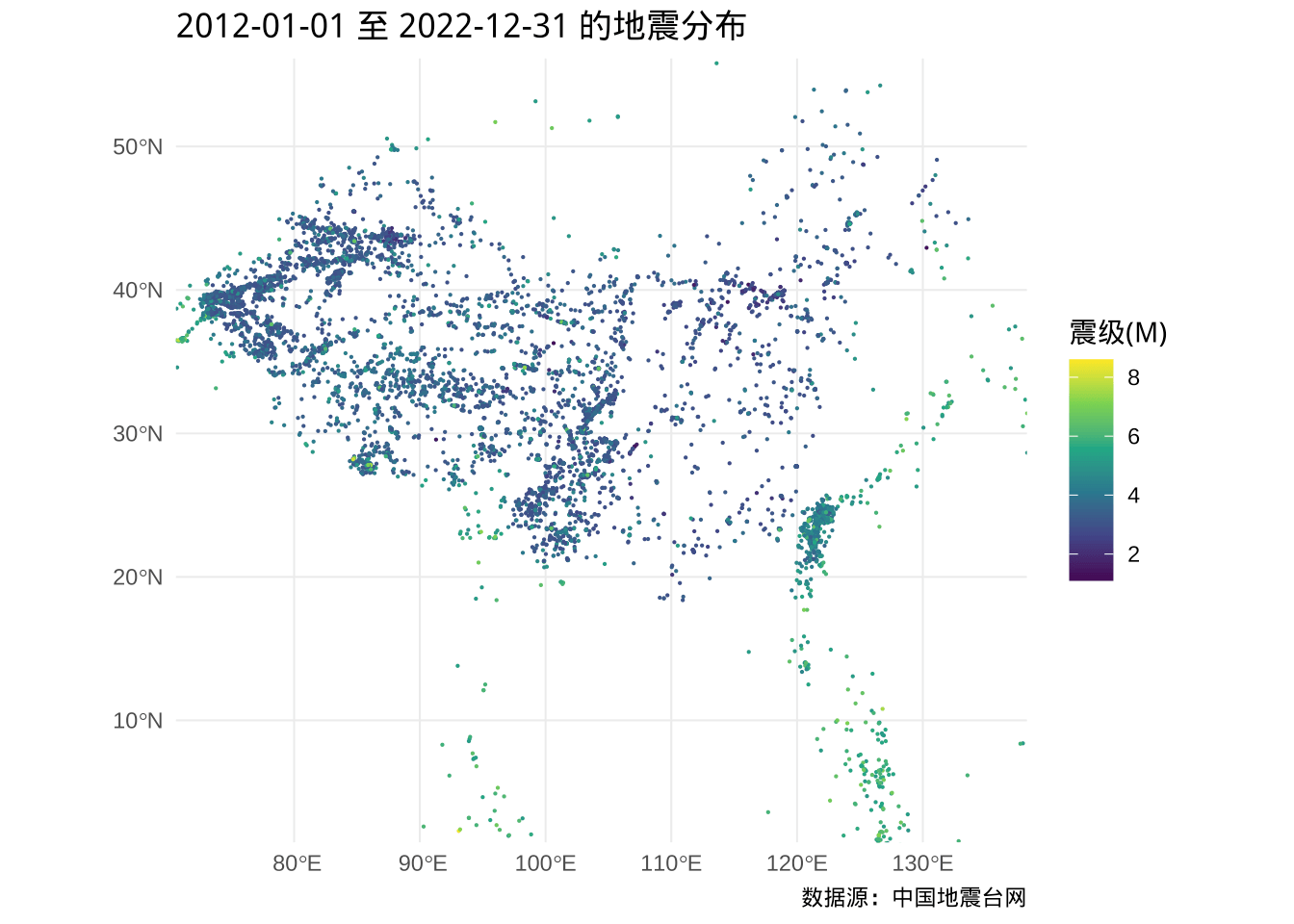ggplot() +
geom_sf(
data = subset(dat_sf, subset = 震级(M) > 4.5),
aes(color = 震级(M)), cex = .5
) +
scale_color_viridis_c() +
coord_sf(xlim = c(73.68, 135.2), ylim = c(3.984, 53.65)) +
theme_minimal() +
labs(
title = "2012-01-01 至 2022-12-31 的地震分布",
caption = "数据源：中国地震台网"
)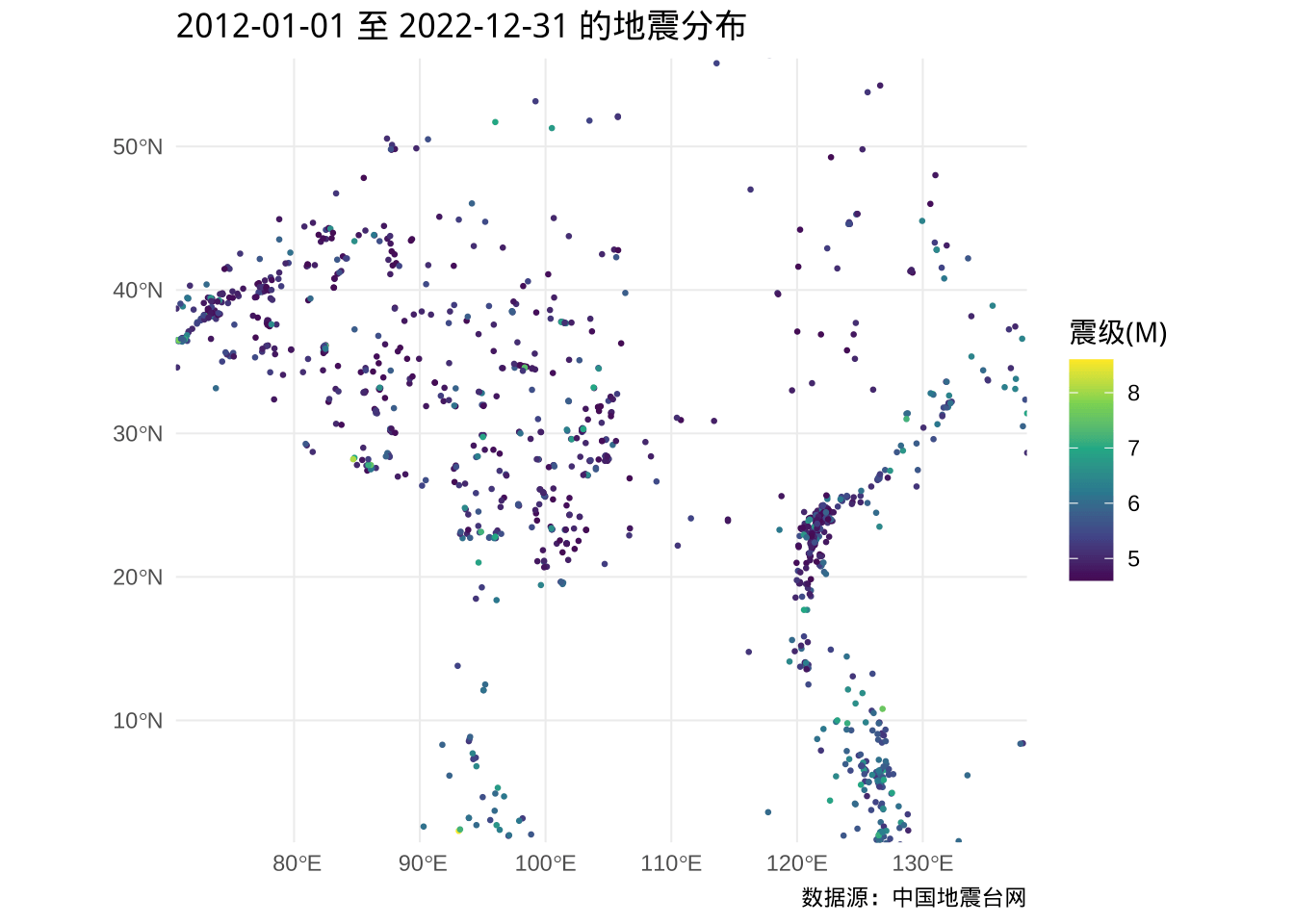# 读取 GeoJSON 格式的多边形矢量地图数据
china_map <- read_sf("data/中华人民共和国.json", as_tibble = FALSE)
china_map
## Simple feature collection with 35 features and 10 fields
## Geometry type: MULTIPOLYGON
## Dimension:     XY
## Bounding box:  xmin: 73.5 ymin: 3.397 xmax: 135.1 ymax: 53.56
## Geodetic CRS:  WGS 84
## First 10 features:
## 1  110000       北京市   <NA>          16 province { "adcode": 100000 }
## 2  120000       天津市   <NA>          16 province { "adcode": 100000 }
## 3  130000       河北省   <NA>          11 province { "adcode": 100000 }
## 4  140000       山西省   <NA>          11 province { "adcode": 100000 }
## 5  150000 内蒙古自治区   <NA>          12 province { "adcode": 100000 }
## 6  210000       辽宁省   <NA>          14 province { "adcode": 100000 }
## 7  220000       吉林省   <NA>           9 province { "adcode": 100000 }
## 8  230000     黑龙江省   <NA>          13 province { "adcode": 100000 }
## 9  310000       上海市   <NA>          16 province { "adcode": 100000 }
## 10 320000       江苏省   <NA>          13 province { "adcode": 100000 }
##    subFeatureIndex        center      centroid acroutes
## 1                0   116.4, 39.9 116.42, 40.19   100000
## 2                1 117.19, 39.13 117.35, 39.29   100000
## 3                2 114.50, 38.05                 100000
## 4                3 112.55, 37.86 112.30, 37.62   100000
## 5                4 111.67, 40.82 114.08, 44.33   100000
## 6                5   123.4, 41.8   122.6, 41.3   100000
## 7                6 125.32, 43.89   126.2, 43.7   100000
## 8                7 126.64, 45.76 127.69, 48.04   100000
## 9                8 121.47, 31.23 121.44, 31.07   100000
## 10               9 118.77, 32.04 119.49, 32.98   100000
##                          geometry
## 1  MULTIPOLYGON (((117.3 40.58...
## 2  MULTIPOLYGON (((117.8 39.4,...
## 3  MULTIPOLYGON (((117.5 40.65...
## 4  MULTIPOLYGON (((110.4 34.6,...
## 5  MULTIPOLYGON (((97.17 42.8,...
## 6  MULTIPOLYGON (((123.5 39.79...
## 7  MULTIPOLYGON (((129.6 42.42...
## 8  MULTIPOLYGON (((123.6 46.22...
## 9  MULTIPOLYGON (((120.9 31.02...
## 10 MULTIPOLYGON (((117.3 34.56...

ggplot() +
geom_sf(data = china_map, color = "gray", fill = NA) +
geom_sf(
data = subset(dat_sf, subset = 震级(M) >= 4.5),
aes(color = 震级(M)), cex = .5
) +
scale_color_viridis_c() +
coord_sf(xlim = c(73.68, 135.2), ylim = c(3.984, 53.65)) +
theme_minimal() +
labs(
title = "2012-01-01 至 2022-12-31 的地震分布",
caption = "数据源：中国地震台网"
)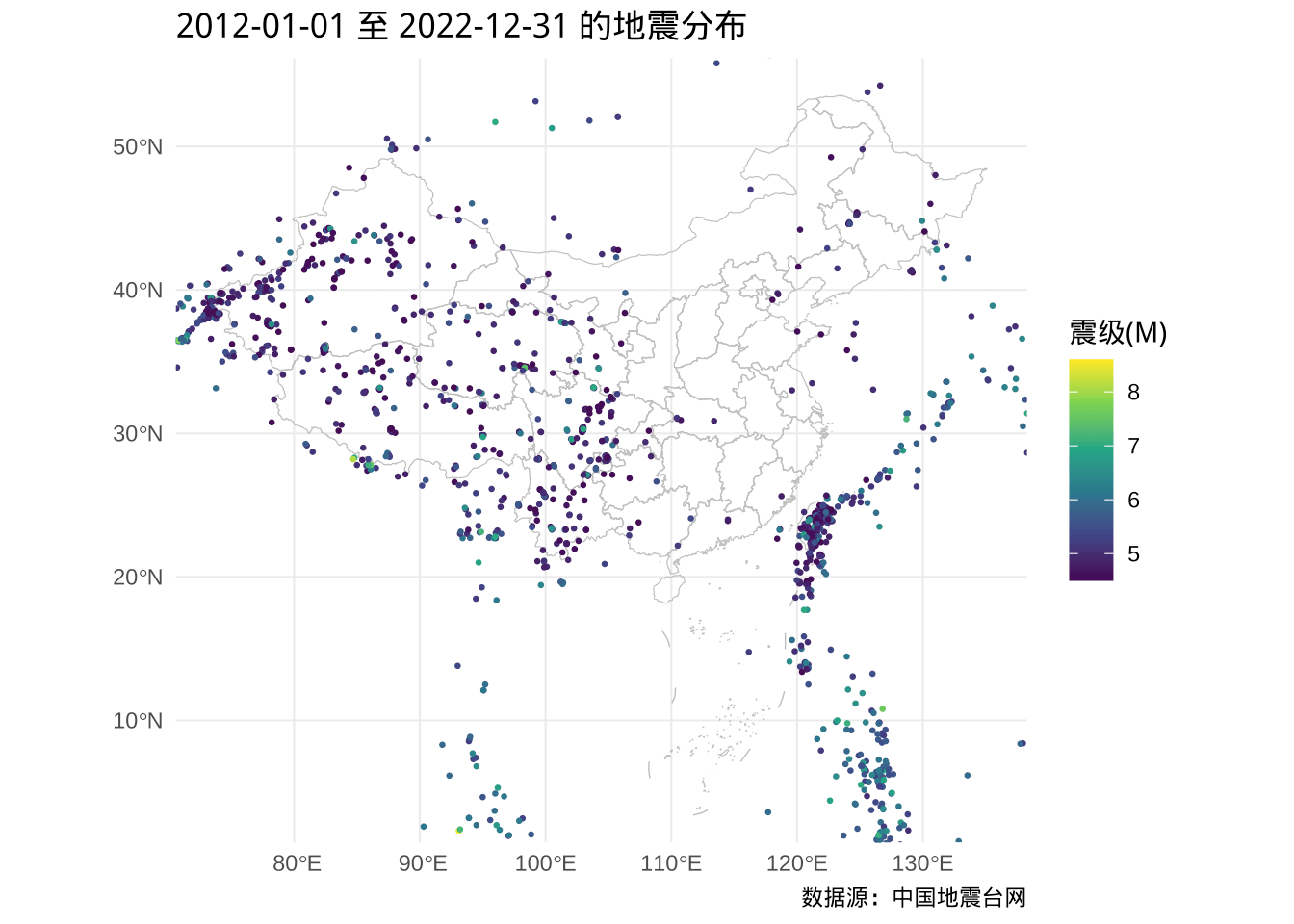ggplot() +
geom_sf(data = china_map, color = "gray", fill = NA) +
geom_sf(data = subset(dat_sf, subset = 震级(M) >= 6.5), aes(color = 震级(M))) +
scale_color_viridis_c() +
coord_sf(xlim = c(73.68, 135.2), ylim = c(3.984, 53.65)) +
theme_minimal() +
labs(
title = "2012-01-01 至 2022-12-31 的地震分布",
caption = "数据源：中国地震台网"
)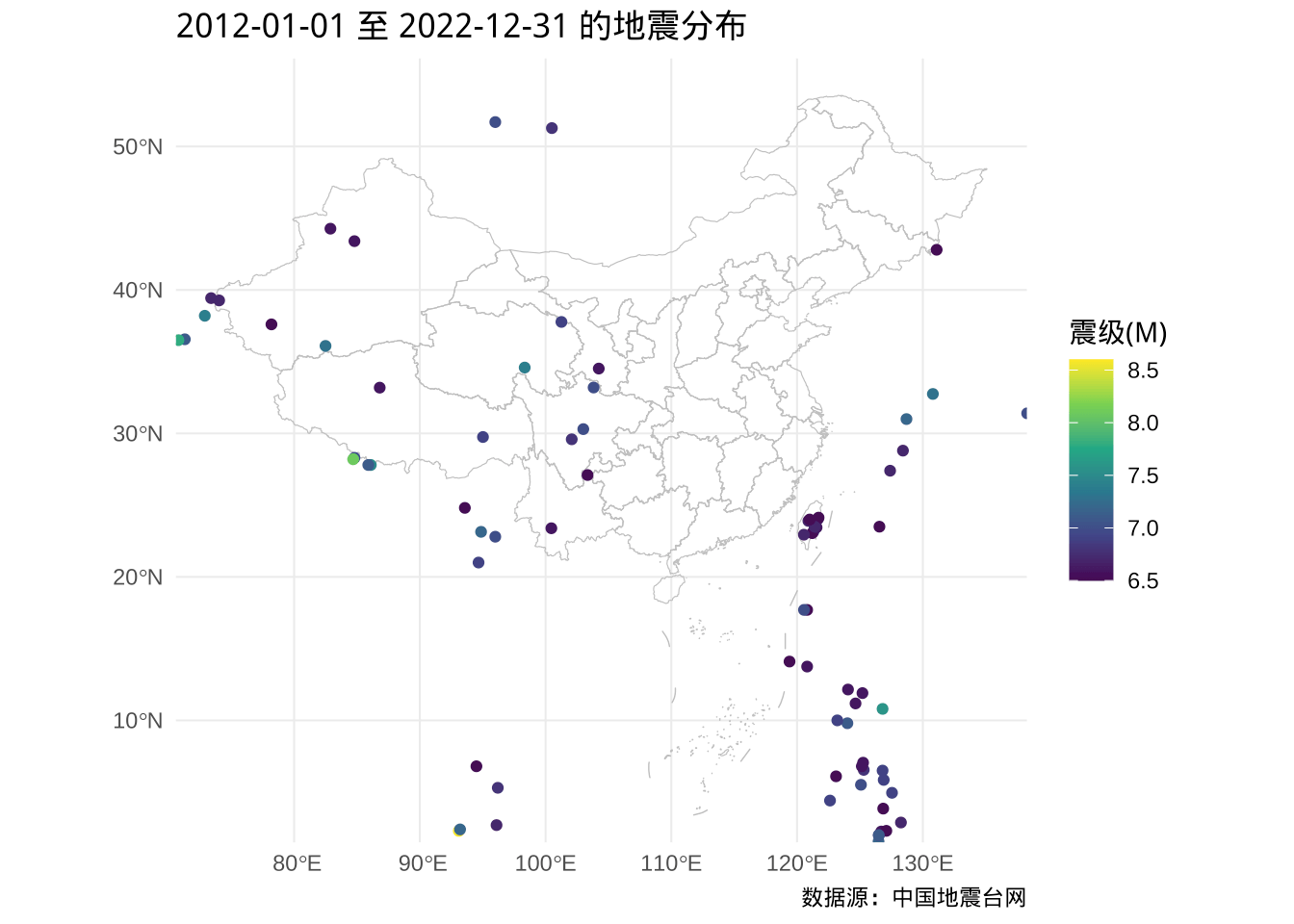china_map_ch <- st_convex_hull(china_map)

china_map_union <- st_union(china_map_ch)

sub_dat_sf <- subset(dat_sf[china_map_union, op = st_within], subset = 震级(M) >= 6.5)
sub_dat_sf
## Simple feature collection with 26 features and 8 fields
## Geometry type: POINT
## Dimension:     XY
## Bounding box:  xmin: 74.04 ymin: 14.1 xmax: 121.7 ymax: 44.27
## Geodetic CRS:  WGS 84
## First 10 features:
##                 发震时刻 震级(M) 深度(千米)         参考位置 时段 月份 年份
## 313  2022-09-18 14:44:14     6.9         10       台湾花莲县   14   09 2022
## 324  2022-09-17 21:41:18     6.5         10       台湾台东县   21   09 2022
## 385  2022-09-05 12:52:18     6.8         16 四川甘孜州泸定县   12   09 2022
## 481  2022-07-27 08:43:27     7.0         10           菲律宾   08   07 2022
## 900  2022-03-23 01:41:38     6.6         20   台湾台东县海域   01   03 2022
## 943  2022-03-14 05:05:50     6.5         10       菲律宾群岛   05   03 2022
## 1165 2022-01-08 01:45:27     6.9         10 青海海北州门源县   01   01 2022
## 1969 2021-05-22 02:04:11     7.4         17 青海果洛州玛多县   02   05 2021
## 2813 2020-07-23 04:07:20     6.6         10 西藏那曲市尼玛县   04   07 2020
## 4257 2019-04-18 13:01:05     6.7         24   台湾花莲县海域   13   04 2019
##            日期            geometry
## 313  2022-09-18 POINT (121.3 23.15)
## 324  2022-09-17 POINT (121.2 23.05)
## 385  2022-09-05 POINT (102.1 29.59)
## 481  2022-07-27  POINT (120.5 17.7)
## 900  2022-03-23 POINT (121.5 23.45)
## 943  2022-03-14  POINT (119.4 14.1)
## 1165 2022-01-08 POINT (101.3 37.77)
## 1969 2021-05-22 POINT (98.34 34.59)
## 2813 2020-07-23 POINT (86.81 33.19)
## 4257 2019-04-18 POINT (121.7 24.02)

ggplot() +
geom_sf(data = china_map, color = "gray", fill = NA) +
geom_sf(data = sub_dat_sf, aes(color = 震级(M))) +
scale_color_viridis_c() +
theme_minimal() +
labs(
title = "2012-01-01 至 2022-12-31 的地震分布",
caption = "数据源：中国地震台网"
)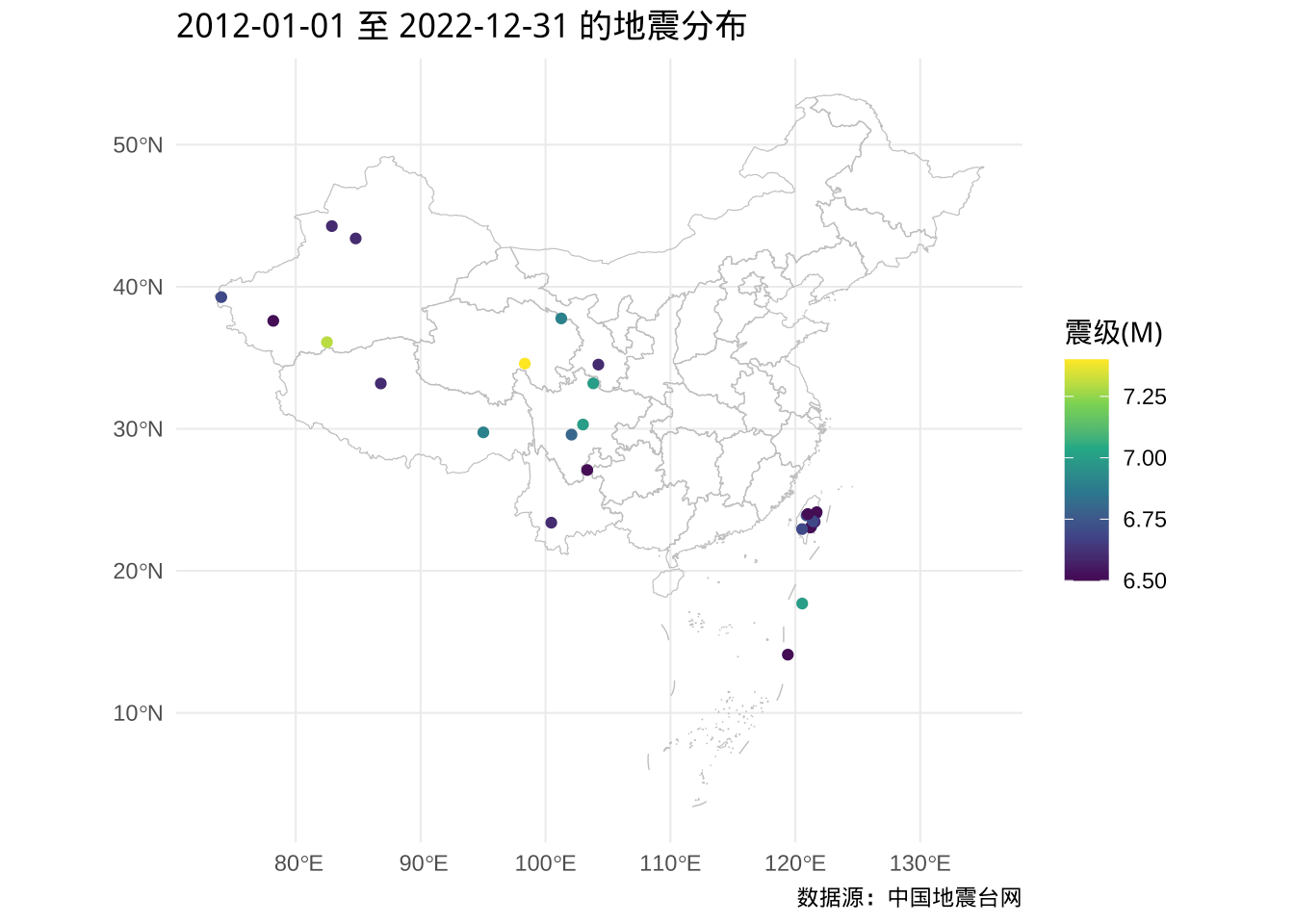### 4.4.2 震次的时段分布

ggplot(data = dat, aes(x = 时段, y = after_stat(count), fill = 年份)) +
geom_bar() +
theme_minimal()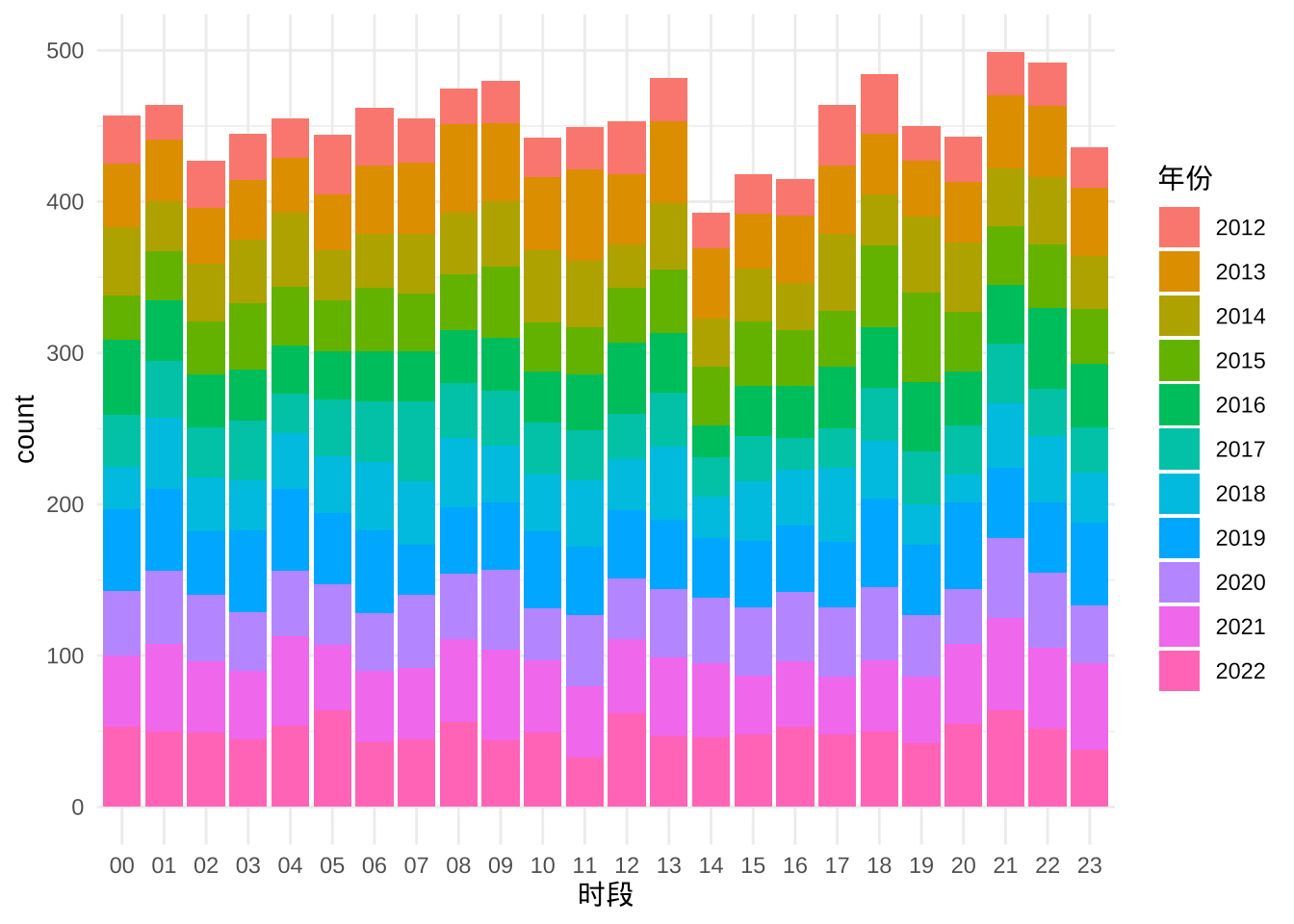ggplot(
data = subset(dat, subset = 震级(M) > 4.5),
aes(
x = 时段, y = after_stat(count),
fill = 年份
)
) +
geom_bar() +
theme_minimal()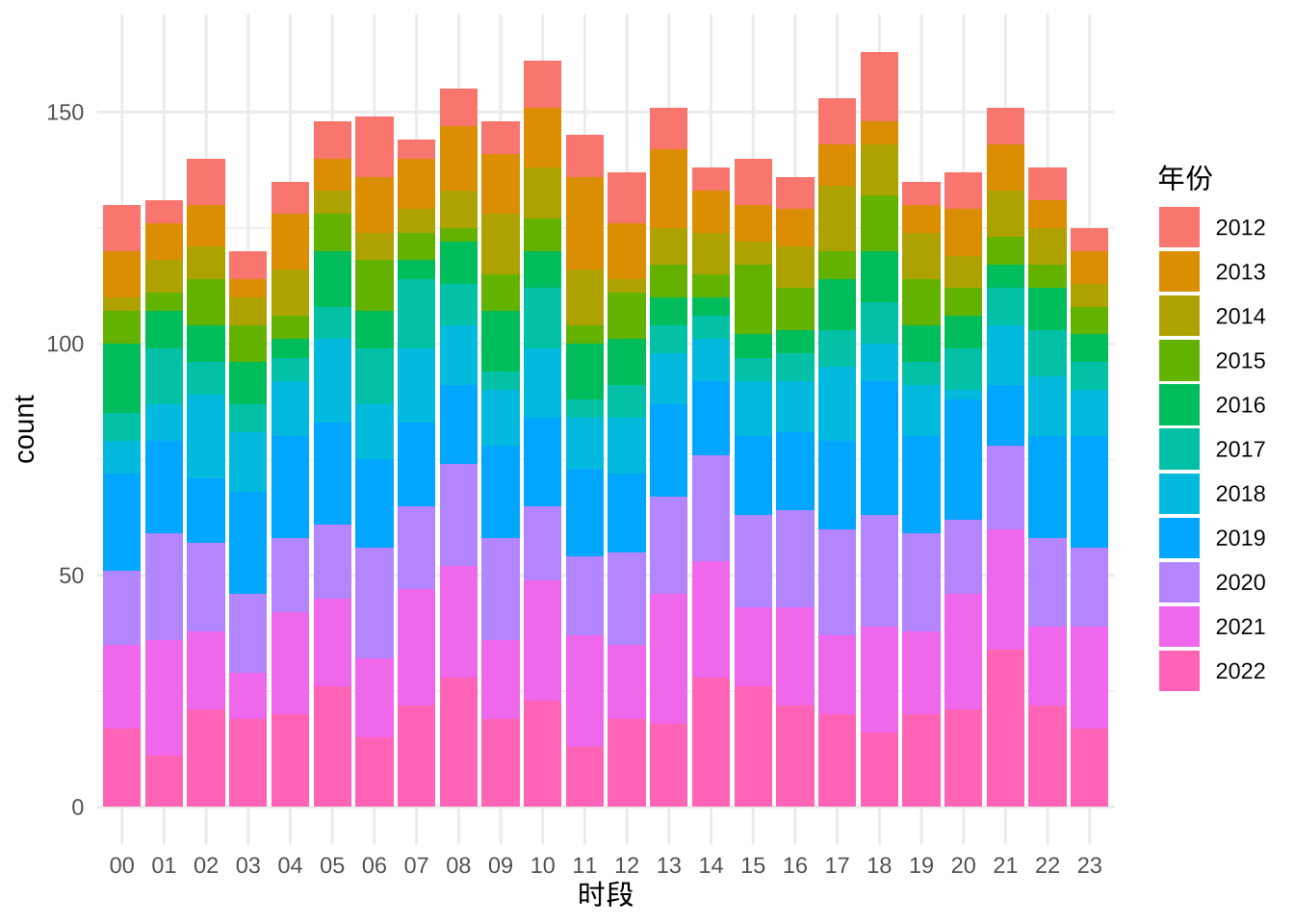ggplot(
data = subset(dat, subset = 震级(M) >= 6),
aes(
x = 时段, y = after_stat(count),
fill = 年份
)
) +
geom_bar() +
theme_minimal()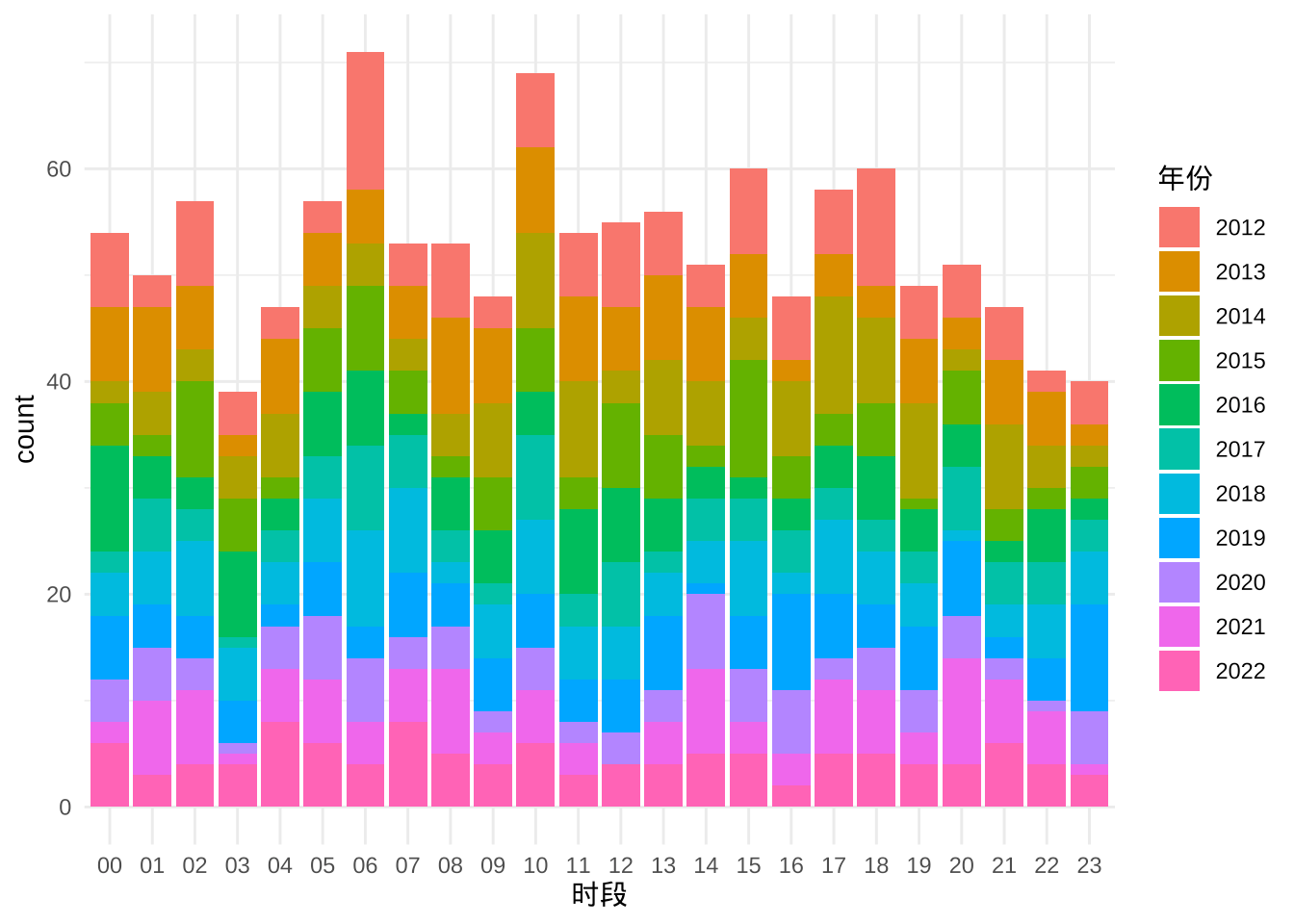### 4.4.3 震级与震深的关系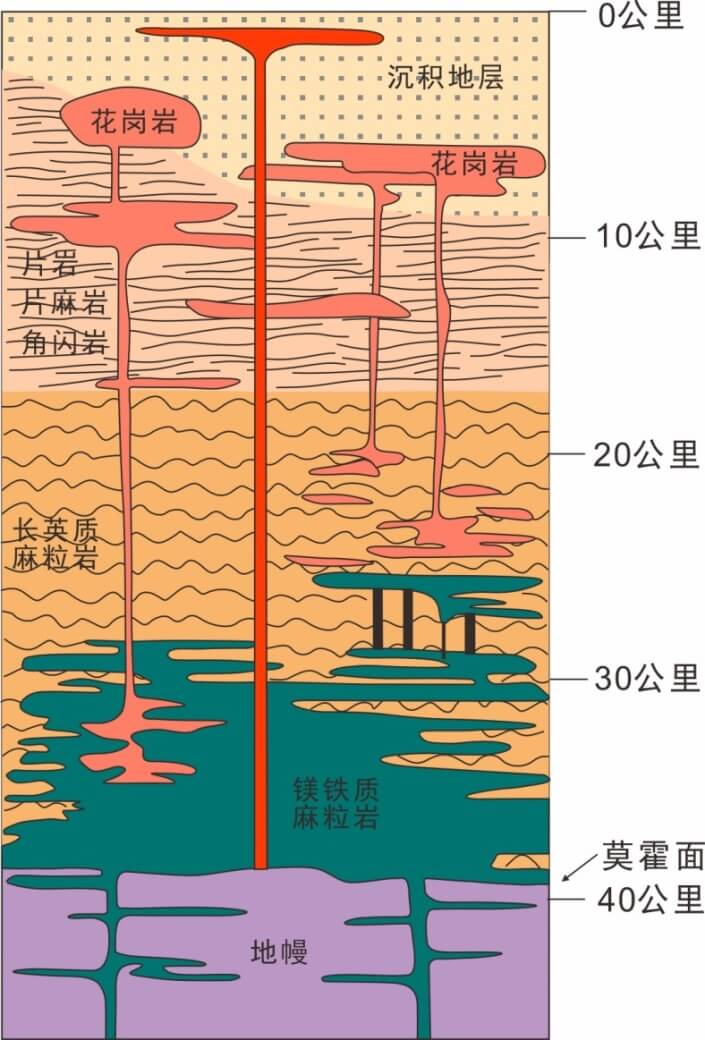4.17来自中国地质调查局网站，展示不同深度下的岩层概况。下图展示震级随震深的关系

ggplot(data = dat, aes(x = 深度(千米), y = 震级(M), color = 年份)) +
geom_point() +
theme_minimal()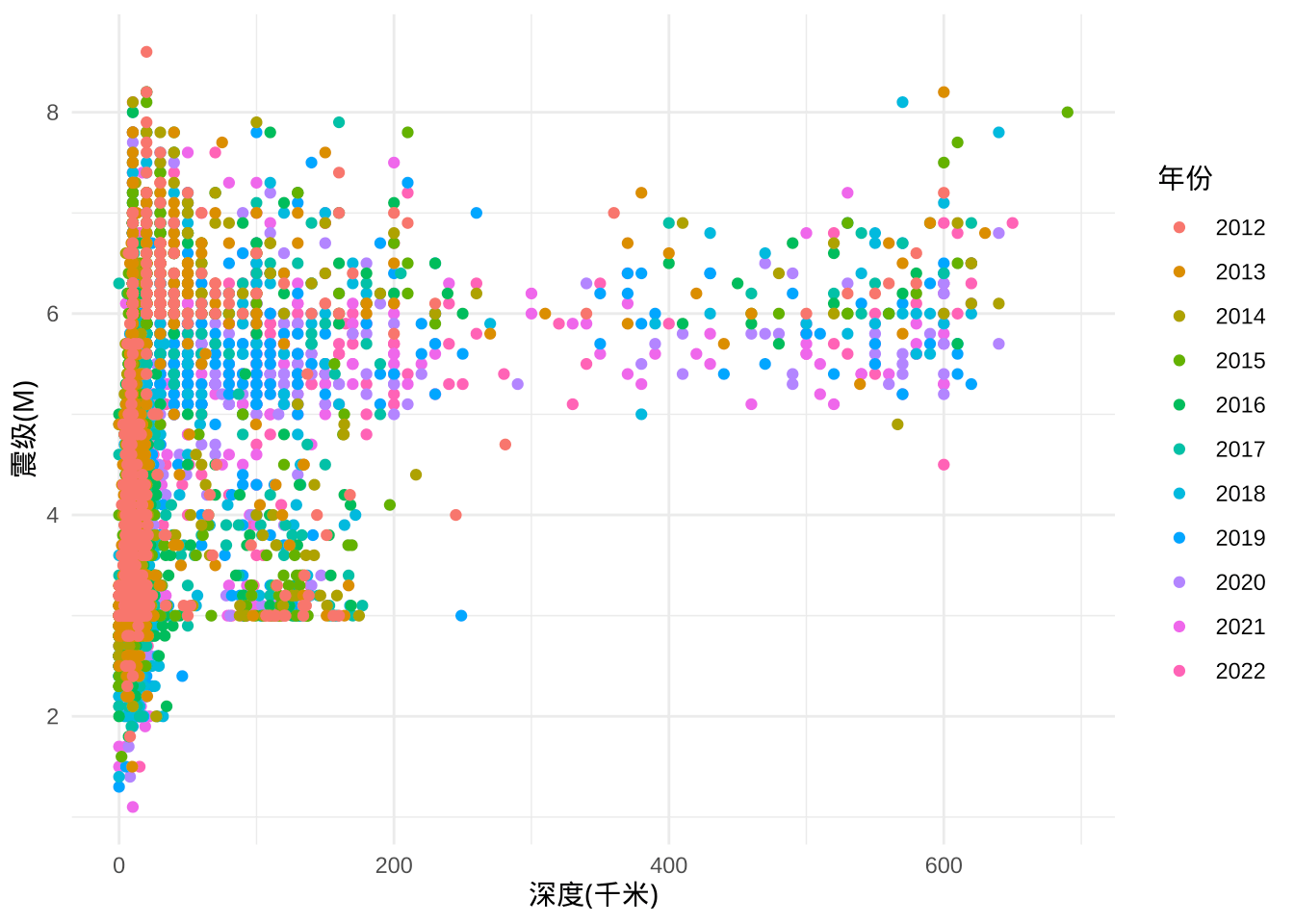subset(dat, subset = 深度(千米) == 0 & 年份 == 2022)
##                 发震时刻 震级(M) 纬度(°) 经度(°) 深度(千米)
## 91   2022-11-27 10:15:35     3.0   38.95   110.3          0
## 273  2022-09-29 00:00:28     3.0   42.73   123.0          0
## 325  2022-09-17 15:20:05     3.0   28.32   111.1          0
## 1106 2022-01-20 13:05:50     2.7   41.87   123.9          0
##                      参考位置 时段 月份 年份       日期
## 91     陕西榆林市神木市(塌陷)   10   11 2022 2022-11-27
## 273  辽宁沈阳市康平县（塌陷）   00   09 2022 2022-09-29
## 325  湖南益阳市安化县（矿震）   15   09 2022 2022-09-17
## 1106 辽宁抚顺市新抚区（矿震）   13   01 2022 2022-01-20

# 5 环境信息

xfun::session_info(packages = c(
"knitr", "rmarkdown", "blogdown",
"ggplot2", "ggbeeswarm", "ggridges",
"sf", "MSG", "showtext"
), dependencies = FALSE)
## R version 4.2.2 (2022-10-31)
## Platform: x86_64-apple-darwin17.0 (64-bit)
## Running under: macOS Big Sur ... 10.16
##
## Locale: en_US.UTF-8 / en_US.UTF-8 / en_US.UTF-8 / C / en_US.UTF-8 / en_US.UTF-8
##
## Package version:
##   blogdown_1.16    ggbeeswarm_0.7.1 ggplot2_3.4.0    ggridges_0.5.4
##   knitr_1.41       MSG_0.8          rmarkdown_2.19   sf_1.0-9
##   showtext_0.9-5
##
## Pandoc version: 2.19.2
##
## Hugo version: 0.109.0

# 6 参考文献

Clarke, Erik, and Scott Sherrill-Mix. 2017. ggbeeswarm: Categorical Scatter (Violin Point) Plots. https://CRAN.R-project.org/package=ggbeeswarm.
Pebesma, Edzer. 2018. Simple Features for R: Standardized Support for Spatial Vector Data.” The R Journal 10 (1): 439–46. https://doi.org/10.32614/RJ-2018-009.
Wickham, Hadley. 2016. ggplot2: Elegant Graphics for Data Analysis. Springer-Verlag New York. https://ggplot2.tidyverse.org.
Wilke, Claus O. 2021. ggridges: Ridgeline Plots in ggplot2. https://CRAN.R-project.org/package=ggridges.
Xie, Yihui, Alison Presmanes Hill, and Amber Thomas. 2017. blogdown: Creating Websites with R Markdown. Boca Raton, Florida: Chapman; Hall/CRC. https://bookdown.org/yihui/blogdown/.